• Java目录监视器源程序 9个目标文件 内容索引:JAVA源码,综合应用,目录监视 用JAVA开发的一个小型的目录监视系统，系统会每5秒自动扫描一次需要监视的目录，可以用来监视目录文件大小及文件增减数目的变化。...java
• 编写多线程程序是为了实现多任务的并发执行，从而能够更好地与用户交互。...(1)Callable规定的方法是call(),Runnable规定的方法是run(). (2)Callable的任务执行后可返回值，而Runnable的任务是不能返回值得 (3...
编写多线程程序是为了实现多任务的并发执行，从而能够更好地与用户交互。一般有四种方法，Thread,Runnable,Callable,使用Executor框架来创建线程池。
Runnable和Callable的区别是， (1)Callable规定的方法是call(),Runnable规定的方法是run(). (2)Callable的任务执行后可返回值，而Runnable的任务是不能返回值得 (3)call方法可以抛出异常，run方法不可以 (4)运行Callable任务可以拿到一个Future对象，表示异步计算的结果。它提供了检查计算是否完成的方法，以等待计算的完成，并检索计算的结果。通过Future对象可以了解任务执行情况，可取消任务的执行，还可获取执行结果。
步骤1：创建实现Runnable接口的类：

class SomeRunnable implements Runnable
{
public void run()
{
//do something here
}
}
步骤2：创建一个类对象：
 Runnable oneRunnable = new SomeRunnable();
  Thread oneThread = new Thread(oneRunnable);
步骤4：启动线程：
  oneThread.start();
至此，一个线程就创建完成了。
该方法执行完成后，线程就消亡了。
public interface Callable<V>
{
V call() throws Exception;
} 
步骤1：创建实现Callable接口的类SomeCallable<Integer>（略）；
步骤2：创建一个类对象：
 Callable<Integer> oneCallable = new SomeCallable<Integer>();
FutureTask<Integer> oneTask = new FutureTask<Integer>(oneCallable);
Thread oneThread = new Thread(oneTask);
步骤5：启动线程：
oneThread.start();
至此，一个线程就创建完成了。
class SomeThead extends Thraad
{
public void run()
{
//do something here
}
}
步骤2：构造子类的一个对象：
     SomeThread oneThread = new SomeThread();
步骤3：启动线程：
 oneThread.start();
至此，一个线程就创建完成了。
注释：这种创建线程的方法不够好，主要是因为其涉及运行机制问题，影响程序性能。

4、使用Executor框架来创建线程池
在Java 5之后，并发编程引入了一堆新的启动、调度和管理线程的API。Executor框架便是Java 5中引入的，其内部使用了线程池机制，它在java.util.concurrent 包下，通过该框架来控制线程的启动、执行和关闭，可以简化并发编程的操作。因此，在Java 5之后，通过Executor来启动线程比使用Thread的start方法更好，除了更易管理，效率更好（用线程池实现，节约开销）外，还有关键的一点：有助于避免this逃逸问题。( this逃逸，  是指在构造函数返回之前，其它线程就持有该对象的引用，调用尚未构造完全的对象的方法，可能引发令人疑惑的错误，应该避免this逃逸事件的发生 )。
Executor框架包括：线程池，Executor，Executors，ExecutorService，CompletionService，Future，Callable等。
Executor接口中之定义了一个方法execute（Runnable command），该方法接收一个Runable实例，它用来执行一个任务，任务即一个实现了Runnable接口的类。ExecutorService接口继承自Executor接口，它提供了更丰富的实现多线程的方法，比如，ExecutorService提供了关闭自己的方法，以及可为跟踪一个或多个异步任务执行状况而生成 Future 的方法。 可以调用ExecutorService的shutdown（）方法来平滑地关闭 ExecutorService，调用该方法后，将导致ExecutorService停止接受任何新的任务且等待已经提交的任务执行完成(已经提交的任务会分两类：一类是已经在执行的，另一类是还没有开始执行的)，当所有已经提交的任务执行完毕后将会关闭ExecutorService。因此我们一般用该接口来实现和管理多线程。
ExecutorService的生命周期包括三种状态：运行、关闭、终止。创建后便进入运行状态，当调用了shutdown（）方法时，便进入关闭状态，此时意味着ExecutorService不再接受新的任务，但它还在执行已经提交了的任务，当素有已经提交了的任务执行完后，便到达终止状态。如果不调用shutdown（）方法，ExecutorService会一直处在运行状态，不断接收新的任务，执行新的任务，服务器端一般不需要关闭它，保持一直运行即可。
Executors提供了一系列工厂方法用于创先线程池，返回的线程池都实现了ExecutorService接口。
创建固定数目线程的线程池。
创建一个可缓存的线程池，调用execute将重用以前构造的线程（如果线程可用）。如果现有线程没有可用的，则创建一个新线   程并添加到池中。终止并从缓存中移除那些已有 60 秒钟未被使用的线程。
创建一个单线程化的Executor。
创建一个支持定时及周期性的任务执行的线程池，多数情况下可用来替代Timer类。

Executor执行Runnable任务
通过Executors的以上四个静态工厂方法获得 ExecutorService实例，而后调用该实例的execute（Runnable command）方法即可。一旦Runnable任务传递到execute（）方法，该方法便会自动在一个线程上
import java.util.concurrent.ExecutorService;
import java.util.concurrent.Executors;

public static void main(String[] args){
for (int i = 0; i < 5; i++){
executorService.execute(new TestRunnable());
System.out.println("************* a" + i + " *************");
}
executorService.shutdown();
}
}

class TestRunnable implements Runnable{
public void run(){
}
}  

某次执行后的结果如下：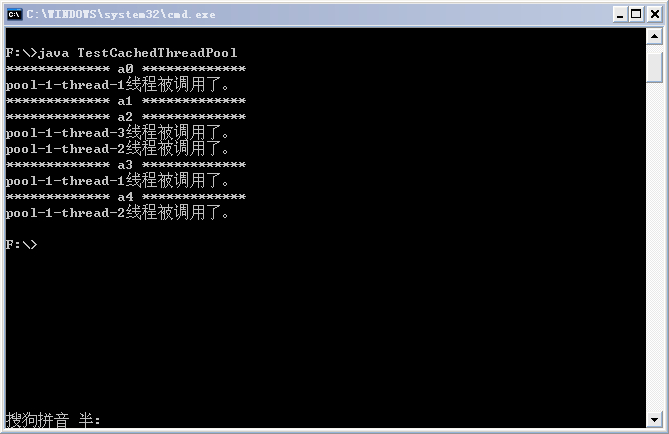Executor执行Callable任务
在Java 5之后，任务分两类：一类是实现了Runnable接口的类，一类是实现了Callable接口的类。两者都可以被ExecutorService执行，但是Runnable任务没有返回值，而Callable任务有返回值。并且Callable的call()方法只能通过ExecutorService的submit(Callable<T> task) 方法来执行，并且返回一个 <T>Future<T>，是表示任务等待完成的 Future。
Callable接口类似于Runnable，两者都是为那些其实例可能被另一个线程执行的类设计的。但是 Runnable 不会返回结果，并且无法抛出经过检查的异常而Callable有返回结果，而且当获取返回结果时可能会抛出异常。Callable中的call()方法类似Runnable的run()方法，区别同样是有返回值，后者没有。
当将一个Callable的对象传递给ExecutorService的submit方法，则该call方法自动在一个线程上执行，并且会返回执行结果Future对象。同样，将Runnable的对象传递给ExecutorService的submit方法，则该run方法自动在一个线程上执行，并且会返回执行结果Future对象，但是在该Future对象上调用get方法，将返回null。
下面给出一个Executor执行Callable任务的示例代码：
import java.util.ArrayList;
import java.util.List;
import java.util.concurrent.*;

public class CallableDemo{
public static void main(String[] args){
List<Future<String>> resultList = new ArrayList<Future<String>>();

//创建10个任务并执行
for (int i = 0; i < 10; i++){
//使用ExecutorService执行Callable类型的任务，并将结果保存在future变量中
//将任务执行结果存储到List中
}

//遍历任务的结果
for (Future<String> fs : resultList){
try{
while(!fs.isDone);//Future返回如果没有完成，则一直循环等待，直到Future返回完成
System.out.println(fs.get());     //打印各个线程（任务）执行的结果
}catch(InterruptedException e){
e.printStackTrace();
}catch(ExecutionException e){
e.printStackTrace();
}finally{
//启动一次顺序关闭，执行以前提交的任务，但不接受新任务
executorService.shutdown();
}
}
}
}

private int id;

this.id = id;
}

/**
* 任务的具体过程，一旦任务传给ExecutorService的submit方法，
* 则该方法自动在一个线程上执行
*/
public String call() throws Exception {
//该返回结果将被Future的get方法得到
}
}  
某次执行结果如下：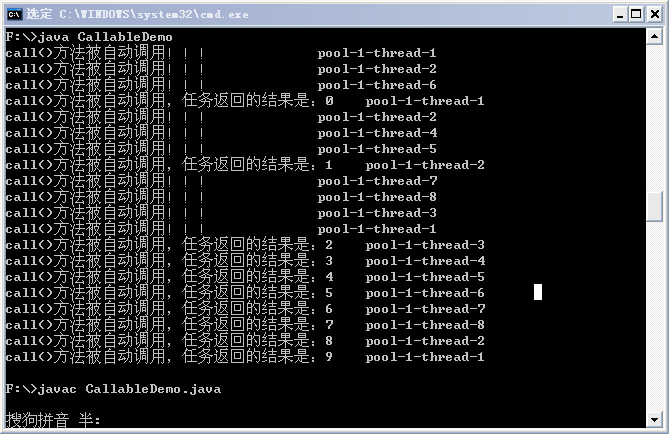从结果中可以同样可以看出，submit也是首先选择空闲线程来执行任务，如果没有，才会创建新的线程来执行任务。另外，需要注意：如果Future的返回尚未完成，则get（）方法会阻塞等待，直到Future完成返回，可以通过调用isDone（）方法判断Future是否完成了返回。

自定义线程池
import java.util.concurrent.ArrayBlockingQueue;
import java.util.concurrent.BlockingQueue;
import java.util.concurrent.TimeUnit;

public static void main(String[] args){
//创建等待队列
BlockingQueue<Runnable> bqueue = new ArrayBlockingQueue<Runnable>(20);
//创建线程池，池中保存的线程数为3，允许的最大线程数为5
//创建七个任务
//每个任务会在一个线程上执行
pool.execute(t1);
pool.execute(t2);
pool.execute(t3);
pool.execute(t4);
pool.execute(t5);
pool.execute(t6);
pool.execute(t7);
//关闭线程池
pool.shutdown();
}
}

@Override
public void run(){
try{
}catch(InterruptedException e){
e.printStackTrace();
}
}
}  
运行结果如下：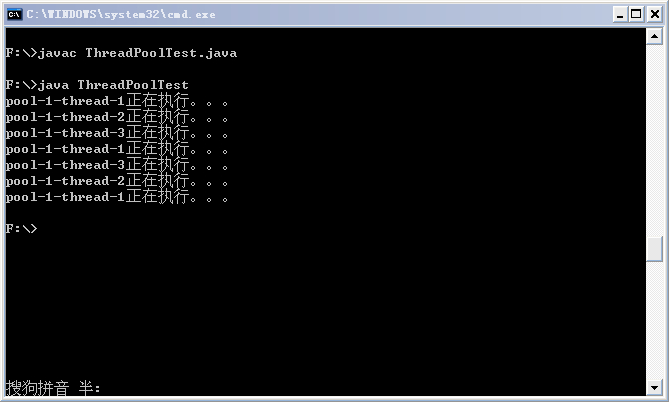public ThreadPoolExecutor (int corePoolSize, int maximumPoolSize, long         keepAliveTime, TimeUnit unit,BlockingQueue<Runnable> workQueue)

corePoolSize：线程池中所保存的线程数，包括空闲线程。
maximumPoolSize：池中允许的最大线程数。
keepAliveTime：当线程数大于核心数时，该参数为所有的任务终止前，多余的空闲线程等待新任务的最长时间。
unit：等待时间的单位。
workQueue：任务执行前保存任务的队列，仅保存由execute方法提交的Runnable任务。
展开全文• ## Java集合面试题

万次阅读 多人点赞 2019-06-25 14:46:19
Java集合面试题 Java 集合框架的基础接口有哪些？ Collection ，为集合层级的根接口。一个集合代表一组对象，...List ，是一个有序集合，可以包含重复元素。你可以通过它的索引来访问任何元素。List 更像长度动态...
Java集合面试题
Java 集合框架的基础接口有哪些？
Collection ，为集合层级的根接口。一个集合代表一组对象，这些对象即为它的元素。Java 平台不提供这个接口任何直接的实现。
Set ，是一个不能包含重复元素的集合。这个接口对数学集合抽象进行建模，被用来代表集合，就如一副牌。List ，是一个有序集合，可以包含重复元素。你可以通过它的索引来访问任何元素。List 更像长度动态变换的数组。 Map ，是一个将 key 映射到 value 的对象。一个 Map 不能包含重复的 key，每个 key 最多只能映射一个 value 。一些其它的接口有 Queue、Dequeue、SortedSet、SortedMap 和 ListIterator 。
? 为何 Collection 不从 Cloneable 和 Serializable 接口继承？
Collection 接口指定一组对象，对象即为它的元素。
如何维护这些元素由 Collection 的具体实现决定。例如，一些如 List 的 Collection 实现允许重复的元素，而其它的如 Set 就不允许。很多 Collection 实现有一个公有的 clone 方法。然而，把它放到集合的所有实现中也是没有意义的。这是因为 Collection 是一个抽象表现，重要的是实现。
当与具体实现打交道的时候，克隆或序列化的语义和含义才发挥作用。所以，具体实现应该决定如何对它进行克隆或序列化，或它是否可以被克隆或序列化。在所有的实现中授权克隆和序列化，最终导致更少的灵活性和更多的限制，特定的实现应该决定它是否可以被克隆和序列化。
为何 Map 接口不继承 Collection 接口？
尽管 Map 接口和它的实现也是集合框架的一部分，但 Map 不是集合，集合也不是 Map。因此，Map 继承 Collection 毫无意义，反之亦然。
如果 Map 继承 Collection 接口，那么元素去哪儿？Map 包含 key-value 对，它提供抽取 key 或 value 列表集合( Collection )的方法，但是它不适合“一组对象”规范。
? 为何 Map 接口不继承 Collection 接口？
尽管 Map 接口和它的实现也是集合框架的一部分，但 Map 不是集合，集合也不是 Map。因此，Map 继承 Collection 毫无意义，反之亦然。
如果 Map 继承 Collection 接口，那么元素去哪儿？Map 包含 key-value 对，它提供抽取 key 或 value 列表集合( Collection )的方法，但是它不适合“一组对象”规范。
? Collection 和 Collections 的区别？
Collection ，是集合类的上级接口，继承与他的接口主要有 Set 和List 。Collections ，是针对集合类的一个工具类，它提供一系列静态方法实现对各种集合的搜索、排序、线程安全化等操作。
? 集合框架里实现的通用算法有哪些？
Java 集合框架提供常用的算法实现，比如排序和搜索。
Collections类包含这些方法实现。大部分算法是操作 List 的，但一部分对所有类型的集合都是可用的。部分算法有排序、搜索、混编、最大最小值。
? 集合框架底层数据结构总结
1）List
ArrayList ：Object 数组。Vector ：Object 数组。LinkedList ：双向链表(JDK6 之前为循环链表，JDK7 取消了循环)。
2）Map
HashMap ：
3）Set
什么是迭代器(Iterator)？
Iterator 接口，提供了很多对集合元素进行迭代的方法。每一个集合类都包含了可以返回迭代器实例的迭代方法。迭代器可以在迭代的过程中删除底层集合的元素，但是不可以直接调用集合的 #remove(Object Obj) 方法删除，可以通过迭代器的 #remove() 方法删除。
? Iterator 和 ListIterator 的区别是什么？
Iterator 可用来遍历 Set 和 List 集合，但是 ListIterator 只能用来遍历 List。Iterator 对集合只能是前向遍历，ListIterator 既可以前向也可以后向。ListIterator 实现了 Iterator 接口，并包含其他的功能。比如：增加元素，替换元素，获取前一个和后一个元素的索引等等。
? 快速失败（fail-fast）和安全失败（fail-safe）的区别是什么？
差别在于 ConcurrentModification 异常：
快速失败：当你在迭代一个集合的时候，如果有另一个线程正在修改你正在访问的那个集合时，就会抛出一个 ConcurrentModification 异常。 在 java.util 包下的都是快速失败。安全失败：你在迭代的时候会去底层集合做一个拷贝，所以你在修改上层集合的时候是不会受影响的，不会抛出 ConcurrentModification 异常。在 java.util.concurrent 包下的全是安全失败的。
? 如何删除 List 中的某个元素？
有两种方式，分别如下：
方式一，使用 Iterator ，顺序向下，如果找到元素，则使用 remove 方法进行移除。方式二，倒序遍历 List ，如果找到元素，则使用 remove 方法进行移除。
? Enumeration 和 Iterator 接口有什么不同？
Enumeration 跟 Iterator 相比较快两倍，而且占用更少的内存。但是，Iterator 相对于 Enumeration 更安全，因为其他线程不能修改当前迭代器遍历的集合对象。同时，Iterators 允许调用者从底层集合中移除元素，这些 Enumerations 都没法完成。
对于很多胖友，可能并未使用过 Enumeration 类，所以可以看看 《Java Enumeration 接口》 文章。
? 为何 Iterator 接口没有具体的实现？
Iterator 接口，定义了遍历集合的方法，但它的实现则是集合实现类的责任。每个能够返回用于遍历的 Iterator 的集合类都有它自己的 Iterator 实现内部类。
这就允许集合类去选择迭代器是 fail-fast 还是 fail-safe 的。比如，ArrayList 迭代器是 fail-fast 的，而 CopyOnWriteArrayList 迭代器是 fail-safe 的。
Comparable 和 Comparator 的区别?
Comparable 接口，在 java.lang 包下，用于当前对象和其它对象的比较，所以它有一个 #compareTo(Object obj) 方法用来排序，该方法只有一个参数。Comparator 接口，在 java.util 包下，用于传入的两个对象的比较，所以它有一个 #compare(Object obj1, Object obj2) 方法用来排序，该方法有两个参数。
详细的，可以看看 《Java 自定义比较器》 文章，重点是如何自己实现 Comparable 和 Comparator 的方法。
? compareTo 方法的返回值表示的意思？
大于 0 ，表示对象大于参数对象。小于 0 ，表示对象小于参数对象等于 0 ，表示两者相等。
? 如何对 Object 的 List 排序？
对 Object[] 数组进行排序时，我们可以用 Arrays#sort(...) 方法。对 List<Object> 数组进行排序时，我们可以用 Collections#sort(...) 方法。
有哪些关于 Java 集合框架的最佳实践？
基于应用的需求来选择使用正确类型的集合，这对性能来说是非常重要的。例如，如果元素的大小是固定的，并且知道优先级，我们将会使用一个 Array ，而不是 ArrayList 。一些集合类允许我们指定他们的初始容量。因此，如果我们知道存储数据的大概数值，就可以避免重散列或者大小的调整。总是使用泛型来保证类型安全，可靠性和健壮性。同时，使用泛型还可以避免运行时的 ClassCastException 异常。在 Map 中使用 JDK 提供的不可变类作为一个 key，这样可以避免 hashcode 的实现和我们自定义类的 equals 方法。应该依照接口而不是实现来编程。返回零长度的集合或者数组，而不是返回一个 null ，这样可以防止底层集合是空的。
区别
List 和 Set 区别？
List，Set 都是继承自 Collection 接口。
List 特点：元素有放入顺序，元素可重复。Set 特点：元素无放入顺序，元素不可重复，重复元素会覆盖掉。

注意：元素虽然无放入顺序，但是元素在 Set 中的位置是有该元素的 hashcode 决定的，其位置其实是固定的。
另外 List 支持 for 循环，也就是通过下标来遍历，也可以用迭代器，但是 Set 只能用迭代，因为他无序，无法用下标来取得想要的值。

Set 和 List 对比：
Set：检索元素效率高，删除和插入效率低，插入和删除不会引起元素位置改变。List：和数组类似，List 可以动态增长，查找元素效率低，插入删除元素效率，因为可能会引起其他元素位置改变。
List 和 Map 区别？
List 是对象集合，允许对象重复。Map 是键值对的集合，不允许 key 重复。
Array 和 ArrayList 有何区别？什么时候更适合用 Array？
Array 可以容纳基本类型和对象，而 ArrayList 只能容纳对象。Array 是指定大小的，而 ArrayList 大小是固定的，可自动扩容。Array 没有提供 ArrayList 那么多功能，比如 addAll、removeAll 和 iterator 等。
尽管 ArrayList 明显是更好的选择，但也有些时候 Array 比较好用，比如下面的三种情况。
1、如果列表的大小已经指定，大部分情况下是存储和遍历它们2、对于遍历基本数据类型，尽管 Collections 使用自动装箱来减轻编码任务，在指定大小的基本类型的列表上工作也会变得很慢。3、如果你要使用多维数组，使用 [][] 比 List 会方便。
? ArrayList
优点：ArrayList 是实现了基于动态数组的数据结构，因为地址连续，一旦数据存储好了，查询操作效率会比较高（在内存里是连着放的）。缺点：因为地址连续，ArrayList 要移动数据，所以插入和删除操作效率比较低。
? 适用场景分析：
当需要对数据进行对随机访问的情况下，选用 ArrayList 。  当需要对数据进行多次增加删除修改时，采用 LinkedList 。

如果容量固定，并且只会添加到尾部，不会引起扩容，优先采用 ArrayList 。
当然，绝大数业务的场景下，使用 ArrayList 就够了。主要是，注意好避免 ArrayList 的扩容，以及非顺序的插入。
? ArrayList 是如何扩容的？
直接看 《ArrayList 动态扩容详解》 文章，很详细。主要结论如下：
如果通过无参构造的话，初始数组容量为 0 ，当真正对数组进行添加时，才真正分配容量。每次按照 1.5 倍（位运算）的比率通过 copeOf 的方式扩容。在 JKD6 中实现是，如果通过无参构造的话，初始数组容量为10，每次通过 copeOf 的方式扩容后容量为原来的 1.5 倍。

重点是 1.5 倍扩容，这是和 HashMap 2 倍扩容不同的地方。

ArrayList 集合加入 1 万条数据，应该怎么提高效率？
ArrayList 的默认初始容量为 10 ，要插入大量数据的时候需要不断扩容，而扩容是非常影响性能的。因此，现在明确了 10 万条数据了，我们可以直接在初始化的时候就设置 ArrayList 的容量！
这样就可以提高效率了~
ArrayList 与 Vector 区别？
ArrayList 和 Vector 都是用数组实现的，主要有这么三个区别：
1、Vector 是多线程安全的，线程安全就是说多线程访问同一代码，不会产生不确定的结果，而 ArrayList 不是。这个可以从源码中看出，Vector 类中的方法很多有 synchronized 进行修饰，这样就导致了 Vector 在效率上无法与 ArrayList 相比。

Vector 是一种老的动态数组，是线程同步的，效率很低，一般不赞成使用。
2、两个都是采用的线性连续空间存储元素，但是当空间不足的时候，两个类的增加方式是不同。  3、Vector 可以设置增长因子，而 ArrayList 不可以。
适用场景分析：
1、Vector 是线程同步的，所以它也是线程安全的，而 ArrayList 是线程无需同步的，是不安全的。如果不考虑到线程的安全因素，一般用 ArrayList 效率比较高。

实际场景下，如果需要多线程访问安全的数组，使用 CopyOnWriteArrayList 。
2、如果集合中的元素的数目大于目前集合数组的长度时，在集合中使用数据量比较大的数据，用 Vector 有一定的优势。

HashMap 和 Hashtable 的区别？

Hashtable 是在 Java 1.0 的时候创建的，而集合的统一规范命名是在后来的 Java2.0 开始约定的，而当时其他一部分集合类的发布构成了新的集合框架。

Hashtable 继承 Dictionary ，HashMap 继承的是 Java2 出现的 Map 接口。2、HashMap 去掉了 Hashtable 的 contains 方法，但是加上了 containsValue 和 containsKey 方法。3、HashMap 允许空键值，而 Hashtable 不允许。【重点】4、HashTable 是同步的，而 HashMap 是非同步的，效率上比 HashTable 要高。也因此，HashMap 更适合于单线程环境，而 HashTable 适合于多线程环境。5、HashMap 的迭代器（Iterator）是 fail-fast 迭代器，HashTable的 enumerator 迭代器不是 fail-fast 的。6、HashTable 中数组默认大小是 11 ，扩容方法是 old * 2 + 1 ，HashMap 默认大小是 16 ，扩容每次为 2 的指数大小。
一般现在不建议用 HashTable 。主要原因是两点：
一是，HashTable 是遗留类，内部实现很多没优化和冗余。二是，即使在多线程环境下，现在也有同步的 ConcurrentHashMap 替代，没有必要因为是多线程而用 Hashtable 。
? Hashtable 的 #size() 方法中明明只有一条语句 “return count;” ，为什么还要做同步？
同一时间只能有一条线程执行固定类的同步方法，但是对于类的非同步方法，可以多条线程同时访问。所以，这样就有问题了，可能线程 A 在执行 Hashtable 的 put 方法添加数据，线程 B 则可以正常调用 #size() 方法读取 Hashtable 中当前元素的个数，那读取到的值可能不是最新的，可能线程 A 添加了完了数据，但是没有对 count++ ，线程 B 就已经读取 count 了，那么对于线程 B 来说读取到的 count 一定是不准确的。
而给 #size() 方法加了同步之后，意味着线程 B 调用 #size() 方法只有在线程 A 调用 put 方法完毕之后才可以调用，这样就保证了线程安全性。
HashSet 和 HashMap 的区别？
Set 是线性结构，值不能重复。HashSet 是 Set 的 hash 实现，HashSet 中值不能重复是用 HashMap 的 key 来实现的。  Map 是键值对映射，可以空键空值。HashMap 是 Map 的 hash 实现，key 的唯一性是通过 key 值 hashcode 的唯一来确定，value 值是则是链表结构。

因为不同的 key 值，可能有相同的 hashcode ，所以 value 值需要是链表结构。

他们的共同点都是 hash 算法实现的唯一性，他们都不能持有基本类型，只能持有对象。

为了更好的性能，Netty 自己实现了 key 为基本类型的 HashMap ，例如 IntObjectHashMap 。

HashSet 和 TreeSet 的区别？
HashSet 是用一个 hash 表来实现的，因此，它的元素是无序的。添加，删除和 HashSet 包括的方法的持续时间复杂度是 O(1) 。TreeSet 是用一个树形结构实现的，因此，它是有序的。添加，删除和 TreeSet 包含的方法的持续时间复杂度是 O(logn) 。
? 如何决定选用 HashMap 还是 TreeMap？
对于在 Map 中插入、删除和定位元素这类操作，HashMap 是最好的选择。然而，假如你需要对一个有序的 key 集合进行遍历， TreeMap 是更好的选择。
基于你的 collection 的大小，也许向 HashMap 中添加元素会更快，再将 HashMap 换为 TreeMap 进行有序 key 的遍历。
HashMap 和 ConcurrentHashMap 的区别？
ConcurrentHashMap 是线程安全的 HashMap 的实现。主要区别如下：
1、ConcurrentHashMap 对整个桶数组进行了分割分段(Segment)，然后在每一个分段上都用 lock 锁进行保护，相对 于Hashtable 的 syn 关键字锁的粒度更精细了一些，并发性能更好。而 HashMap 没有锁机制，不是线程安全的。

JDK8 之后，ConcurrentHashMap 启用了一种全新的方式实现,利用 CAS 算法。
2、HashMap 的键值对允许有 null ，但是 ConCurrentHashMap 都不允许。
队列和栈是什么，列出它们的区别？
栈和队列两者都被用来预存储数据。
java.util.Queue 是一个接口，它的实现类在Java并发包中。队列允许先进先出（FIFO）检索元素，但并非总是这样。Deque 接口允许从两端检索元素。栈与队列很相似，但它允许对元素进行后进先出（LIFO）进行检索。
Stack 是一个扩展自 Vector 的类，而 Queue 是一个接口。
原理
HashMap 的工作原理是什么？
我们知道在 Java 中最常用的两种结构是数组和模拟指针（引用），几乎所有的数据结构都可以利用这两种来组合实现，HashMap 也是如此。实际上 HashMap 是一个**“链表散列”**。
HashMap 是基于 hashing 的原理。
HashMap 图解
我们使用 #put(key, value) 方法来存储对象到 HashMap 中，使用 get(key) 方法从 HashMap 中获取对象。当我们给 #put(key, value) 方法传递键和值时，我们先对键调用 #hashCode() 方法，返回的 hashCode 用于找到 bucket 位置来储存 Entry 对象。
? 当两个对象的 hashCode 相同会发生什么？
因为 hashcode 相同，所以它们的 bucket 位置相同，“碰撞”会发生。
因为 HashMap 使用链表存储对象，这个 Entry（包含有键值对的 Map.Entry 对象）会存储在链表中。
? hashCode 和 equals 方法有何重要性？
HashMap 使用 key 对象的 #hashCode() 和 #equals(Object obj) 方法去决定 key-value 对的索引。当我们试着从 HashMap 中获取值的时候，这些方法也会被用到。
如果这两个方法没有被正确地实现，在这种情况下，两个不同 Key 也许会产生相同的 #hashCode() 和 #equals(Object obj) 输出，HashMap 将会认为它们是相同的，然后覆盖它们，而非把它们存储到不同的地方。
同样的，所有不允许存储重复数据的集合类都使用 #hashCode() 和 #equals(Object obj) 去查找重复，所以正确实现它们非常重要。#hashCode() 和 #equals(Object obj) 方法的实现，应该遵循以下规则：
如果 o1.equals(o2) ，那么 o1.hashCode() == o2.hashCode() 总是为 true 的。如果 o1.hashCode() == o2.hashCode() ，并不意味 o1.equals(o2) 会为 true 。
? HashMap 默认容量是多少？
默认容量都是 16 ，负载因子是 0.75 。就是当 HashMap 填充了 75% 的 busket 是就会扩容，最小的可能性是（16 * 0.75 = 12），一般为原内存的 2 倍。
? 有哪些顺序的 HashMap 实现类？
LinkedHashMap ，是基于元素进入集合的顺序或者被访问的先后顺序排序。TreeMap ，是基于元素的固有顺序 (由 Comparator 或者 Comparable 确定)。
? 我们能否使用任何类作为 Map 的 key？
我们可以使用任何类作为 Map 的 key ，然而在使用它们之前，需要考虑以下几点：
1、如果类重写了 equals 方法，它也应该重写 hashcode 方法。  2、类的所有实例需要遵循与 equals 和 hashcode 相关的规则。  3、如果一个类没有使用 equals ，你不应该在 hashcode 中使用它。  4、用户自定义 key 类的最佳实践是使之为不可变的，这样，hashcode 值可以被缓存起来，拥有更好的性能。不可变的类也可以确保hashcode 和 equals 在未来不会改变，这样就会解决与可变相关的问题了。

比如，我有一个 类MyKey ，在 HashMap 中使用它。代码如下：
//传递给MyKey的name参数被用于equals()和hashCode()中
MyKey key = new MyKey('Pankaj'); //assume hashCode=1234
myHashMap.put(key, 'Value');
// 以下的代码会改变key的hashCode()和equals()值
key.setName('Amit'); //assume new hashCode=7890
//下面会返回null，因为HashMap会尝试查找存储同样索引的key，而key已被改变了，匹配失败，返回null
myHashMap.get(new MyKey('Pankaj'));


那就是为何 String 和 Integer 被作为 HashMap 的 key 大量使用。
? HashMap 的长度为什么是 2 的幂次方？
为了能让 HashMap 存取高效，尽量较少碰撞，也就是要尽量把数据分配均匀，每个链表/红黑树长度大致相同。这个实现就是把数据存到哪个链表/红黑树中的算法。
这个算法应该如何设计呢？我们首先可能会想到采用 % 取余的操作来实现。但是，重点来了：
取余(%)操作中如果除数是 2 的幂次则等价于与其除数减一的与(&)操作（也就是说 hash % length == hash & (length - 1) 的前提是 length 是 2 的 n 次方；）。并且，采用二进制位操作 &，相对于 % 能够提高运算效率，
这就解释了 HashMap 的长度为什么是 2 的幂次方。
HashSet 的工作原理是什么？
HashSet 是构建在 HashMap 之上的 Set hashing 实现类。让我们直接撸下源码，代码如下：
// HashSet.java

private transient HashMap<E,Object> map;

private static final Object PRESENT = new Object();


map 属性，当我们创建一个 HashMap 对象时，其内部也会创建一个 map 对象。后续 HashSet 所有的操作，实际都是基于这个 map 之上的封装。  PRESENT 静态属性，所有 map 中 KEY 对应的值，都是它，避免重复创建。  OK ，再来看一眼 add 方法，代码如下： // HashSet.java

return map.put(e, PRESENT) == null;
}


是不是一目了然。
? HashSet 如何检查重复？

艿艿：正如我们上面看到 HashSet 的实现原理，我们自然可以推导出，HashMap 也是如何检查重复滴。

当你把对象加入 HashSet 时，HashSet会先计算对象的hashcode值来判断对象加入的位置，同时也会与其他加入的对象的hashcode值作比较。
如果没有相符的 hashcode ，HashSet会假设对象没有重复出现。但是如果发现有相同 hashcode 值的对象，这时会调用 equals 方法来检查 hashcode 相等的对象是否真的相同。
如果两者相同，HashSet 就不会让加入操作成功。如果两者不同，HashSet 就会让加入操作成功。
【详细可以查看java基础系列】
展开全文java集合面试题
• 看了很多网上关于Qt多线程的应用实例文章，没有几篇真正讲明白Qt多线程原理的。后来发现知乎上有叫“万丈高楼平地起”的作者写的两篇： 《Qt 多线程编程之敲开 QThread 类的大门》 ...
了很多网上关于Qt多线程的应用实例文章，没有几篇真正讲明白Qt多线程原理的。后来发现知乎上有个叫“万丈高楼平地起”的作者写的两篇： 《Qt 多线程编程之敲开 QThread 类的大门》 https://zhuanlan.zhihu.com/p/53270619 《Qt 中的多线程技术》  https://zhuanlan.zhihu.com/p/52612180 再加上官方的说明文档和例程，基本搞清楚了，在官方例程的基础上进行了修改，也供各位Qt学习者参考。本文也参考了以上两篇文章的部分内容。针对官方推荐的第二中方法（moveToThread）实现多线程。
重点概念
先讲几个重点。详细理论请参考以上两篇文章：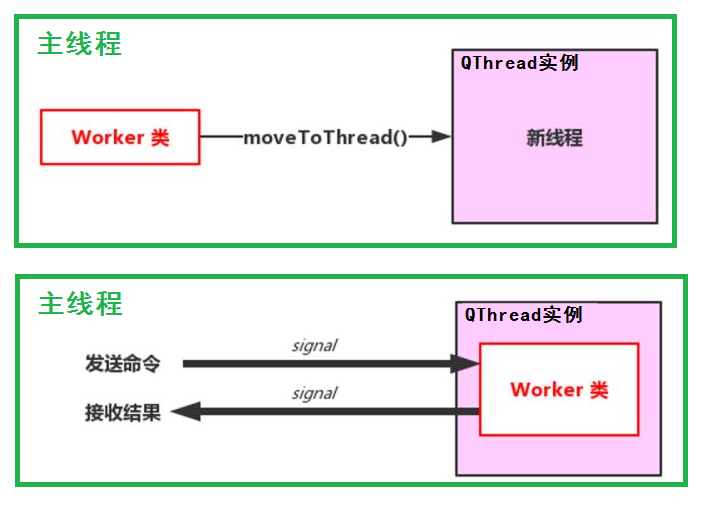实验目的
本次实验中首先在main函数中实现了一个简单的命令交互终端（while循环，用户可以发送命令控制各个线程的启动start、停止stop和检查check。在main函数中创建两个线程容器（controller1和controller2），每个线程中需要做的具体事情由on_doSomething（）函数实现。（本次实验中，要做的事就是不停地让变量count累加1）。
实验过程
（1）创建 基于QObject 的子类 Worker，这个类有个成员函数 on_doSomething()，假如该函数中运行的代码非常耗时。因此需要将这个类对象“移动”到新线程里。这里将on_doSomething放到Workerloop函数中的while()中。通过执行startWorker函数，启动循环体。这样“”耗时工作“” 就在新线程中运行了。Workerloop中的循环体会根据成员变量isStop值来判断是否中止。 Worker 类需要有个槽函数用于执行外界的命令，还需要有个信号来向外界发送结果。在主线程里有个 signal 信号来关联并触发startWorker函数（这里没有使用信号-槽机制改变isStop的值，而是直接在主线程中调用closeWorker函数）。与此同时 Worker 类中有个 signal 信号（resultReady）用于向外界发送运行的结果（本次实验中main函数中存在命令交互while（）,因此运行过程中无法实时显示，只能在命令交互结束后显示，因此没有使用）。如下列代码：
#ifndef WORKER_H
#define WORKER_H
#include <QObject>

class Worker : public QObject
{
Q_OBJECT
public:
explicit Worker( QString name,QObject *parent = nullptr);
signals:
public slots:
void startWorker();
void closeWorker();
private:
void Workerloop();//事件循环
void on_doSomething();//耗时操作
private:
bool isStop;
int count;
QString m_name;
};
#endif // WORKER_H

为了体现是在不同线程中执行的，我们在各个函数中打印当前线程 ID。
#include "worker.h"
#include <QDebug>

Worker::Worker(QString name,QObject *parent) : QObject(parent)
{
m_name =  name;
isStop=false;
count = 0;
}
void Worker::startWorker()
{
isStop = false;
Workerloop();
}
void Worker::closeWorker()
{
isStop = true;
}
void Worker::Workerloop()
{
while(!isStop)
{
on_doSomething();
}
}
void Worker::on_doSomething()
{
count++;
/*   QString workmsg;
workmsg.sprintf("conut=: %d",count);
}

#ifndef CONTROLLER_H
#define CONTROLLER_H

#include <QObject>
#include "worker.h"

class Controller : public QObject
{
Q_OBJECT
public:
explicit Controller(int ctrlId,QString workname,QObject *parent = nullptr);
~Controller();
void start();
void stop();
signals:

public slots:
public:
bool isRunning;
private:
Worker *m_worker;//线程中的对象 new在堆上，需用deleteLater().
int m_ctrlId;
QString m_workname;
};
#endif // CONTROLLER_H

QThread 类的实例通过调用 start() 函数发出的started信号就可以启动函数在新线程中运行。 正常的退出线程其实质是退出事件循环，即执行 exit(int returnCode = 0) 函数。返回0代表成功，其他非零值代表异常。quit() 函数等价于 exit(0)。线程退出后会发出 finished() 信号。
#include "controller.h"
#include <QDebug>
Controller::Controller(int ctrlId,QString workname,QObject *parent) : QObject(parent)
{
m_ctrlId = ctrlId;
m_workname = workname;
isRunning = false;
m_worker = new Worker(workname);

}
Controller::~Controller()
{
{
m_worker->closeWorker();
}
}
void Controller::start()
{
isRunning = true;
}
void Controller::stop()
{
{
m_worker->closeWorker();
isRunning = false;
}
}
{
qDebug()<< str;
}

#include <QCoreApplication>
#include <QDebug>
#include <controller.h>

enum CTRID{
Thrd0,
Thrd1
};
int main(int argc, char *argv[])
{
QCoreApplication a(argc, argv);

QString workername1("Tom");
QString workername2("Jerry");
Controller controller1(Thrd0,workername1);
Controller controller2(Thrd1,workername2);

qDebug()<<"you can enter the following commands by tapping the keyboard:\n";
qDebug()<<"\tstart1\n\tstart2\n\tstop1\n\tstop2\n\tcheck1\n\tcheck2\n\teixt\n";

QTextStream qin(stdin);
QString cmd;
while(1)
{
qin>>cmd;

if(cmd=="exit")
{
controller1.stop();
controller2.stop();
qDebug()<<"main had exited.you can close this console. "<<endl;
break;
}
if(cmd=="check1")
qDebug()<<"controller1 status is: "<<controller1.isRunning<<endl;
if(cmd=="check2")
qDebug()<<"controller2 status is: "<<controller2.isRunning<<endl;
if(cmd=="start1")
{
controller1.start();
qDebug()<<"controller1 is started"<<endl;
}
if(cmd=="start2")
{
controller2.start();
qDebug()<<"controller2 is started"<<endl;
}
if(controller1.isRunning==true)
{
if(cmd=="stop1")
{
controller1.stop();
qDebug()<<"controller1 is stoped"<<endl;
}
}
if(controller2.isRunning==true)
{
if(cmd=="stop2")
{
controller2.stop();
qDebug()<<"controller2 is stoped"<<endl;
}
}
}
return a.exec();
}

看看执行效果：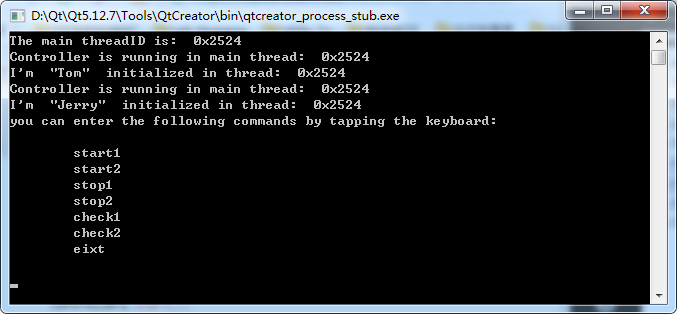启动后，在main函数中完成Controller的实例化和Worker的实例化，因此，Controller1、Controller2、Worker1和Worker2均在存在于主线程0x2524中。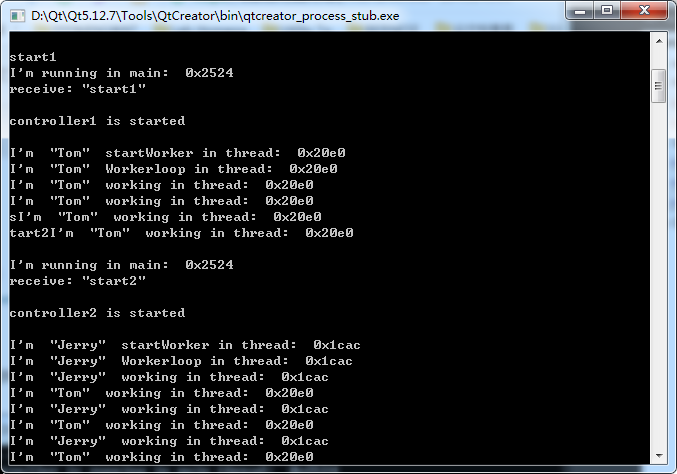分别输入“start1”、“start2”命令，可以看出，两个线程中的Worker分别工作在0x20e0和0x1cac两个线程中。 分别输入“stop1”、“stop2”命令，可以中止两个线程。 说明：中止线程后，再次输入start1、start2，虽然线程能启动，但controller无法执行构造函数，Worker无法启动，因此不能输出信息。
源代码已经上传，可免费下载。 https://download.csdn.net/download/SmartTiger_CSL/12158072 本来这个源代码资源是让大家免费下载的，可是CSDN平台强制改成了需要积分才能下载，因此，这里给出百度网盘免费下载地址 链接1：https://pan.baidu.com/s/1TWvURe7syAVBSaQd8EpTMg 提取码：6d7z链接2：https://pan.baidu.com/s/1tq5oUv1e09VUCxEg1C4xMQ 提取码：qm4g
展开全文• 1. 这本书对Python的知识点的描述很详细，而且排版看的很舒服. 2. 几例题：假装自己从零开始学，将一些有代表性、有意思的例题抽取出来. 3. 还有自己对部分课后复习题，全部课后上机实践题的解题思路
（还在更新中…） 这篇博客花费了我的大量时间和精力，从创作到维护；若认可本篇博客，希望给一个点赞、收藏
并且，遇到了什么问题，请在评论区留言，我会及时回复的

这本书对Python的知识点的描述很详细，而且排版看的很舒服

几个例题： 假装自己从零开始学，将一些有代表性、有意思的例题抽取出来部分复习题： 遇到有意思的复习题，我会拿出来，并且进行分析上机实践： 全部上机实践题的解题思路

文章目录
第一章 Python概述几个例题
第二章 Python语言基础选择题：1、3、7、8思考题：9上机实践：2~6案例研究：使用Pillow库处理图像文件
第三章 程序流程控制几个例题选择题：1、2、3填空题：6思考题：3~6上机实践：2~14案例研究：使用嵌套循环实现图像处理算法
第四章 常用内置数据类型几个例题选择题：11填空题：4、7、8、9、10、13、21思考题：5上机实践：2~14案例研究：科学计算和数据分析
第五章 序列数据类型几个例题选择题：4、5、7、11、12填空题：1、5、6、12、13、14思考题：2、3、5上机实践：2~6案例研究：猜单词游戏
第六章 输入和输出几个例题填空题：1、2例题及上机实践：2~5案例研究：21点扑克牌游戏
第七章 错误和异常处理几个例题案例研究：使用调试器调试Python程序
第八章 函数和函数式编程一些知识点总结和几个例题选择题：1~5思考题：4~11上机实践：2~5案例研究：井字棋游戏
第九章 面向对象的程序设计第十章 模块和客户端第十一章 算法与数据结构基础第十二章 图形用户界面案例研究：简易图形用户界面计算器
第十三章 图形绘制图形绘制模块：tkinter图形绘制模块：turtle
第十四章 数值日期和时间处理第十五章 字符串和文本处理第十六章 文件和数据交换第十七章 数据访问第十八章 网络编程和通信第十九章 并行计算：进程、线程和协程第二十章 系统管理

第一章 Python概述

几个例题
一：Python3.7.4下载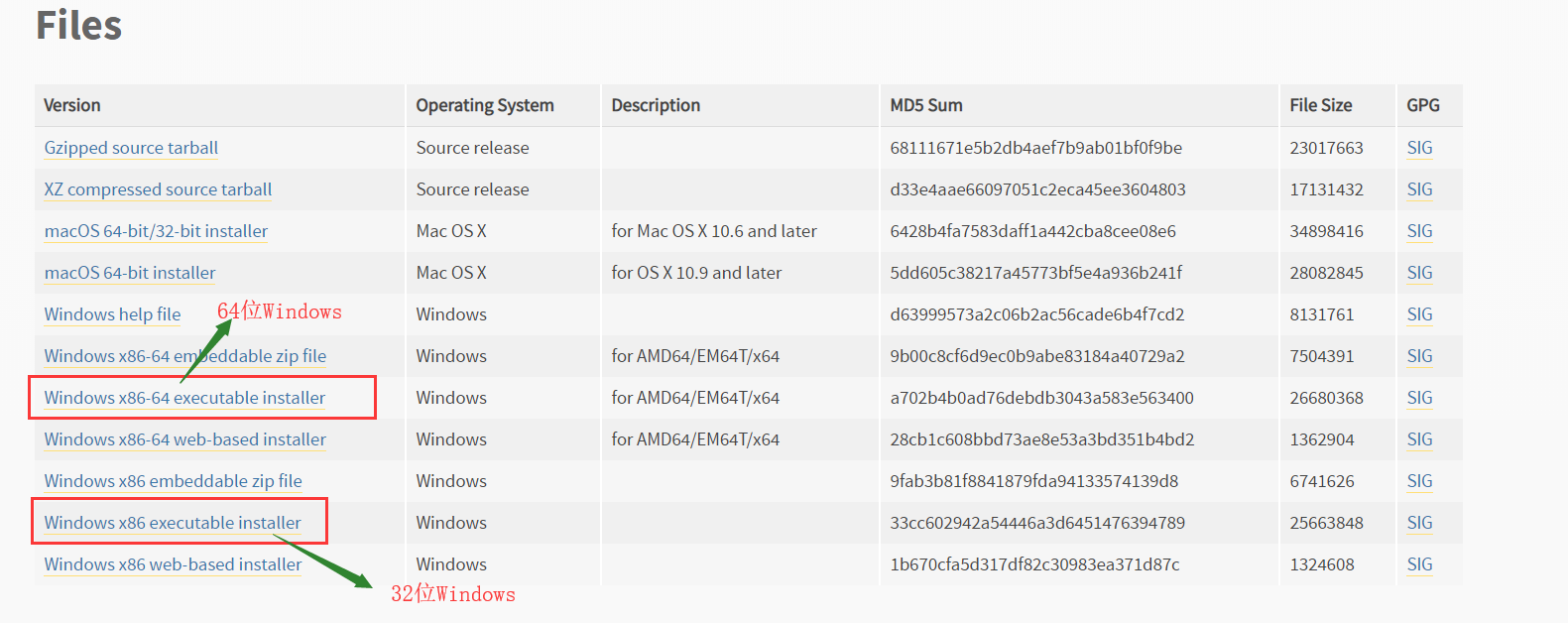下载，安装完python后：出现的四个玩意：Python 3.7 Module Docs，IDLE，Python 3.7 Manuals，Python 3.7（64-bit）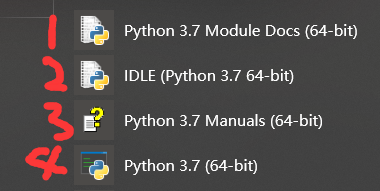Python 3.7 Module Docs（64-bit） 点击之后，会出现一个网页（将我下载的Python3.7.4文件夹中包含的模块都列了出来,页面不止这么点，还可以往下拉）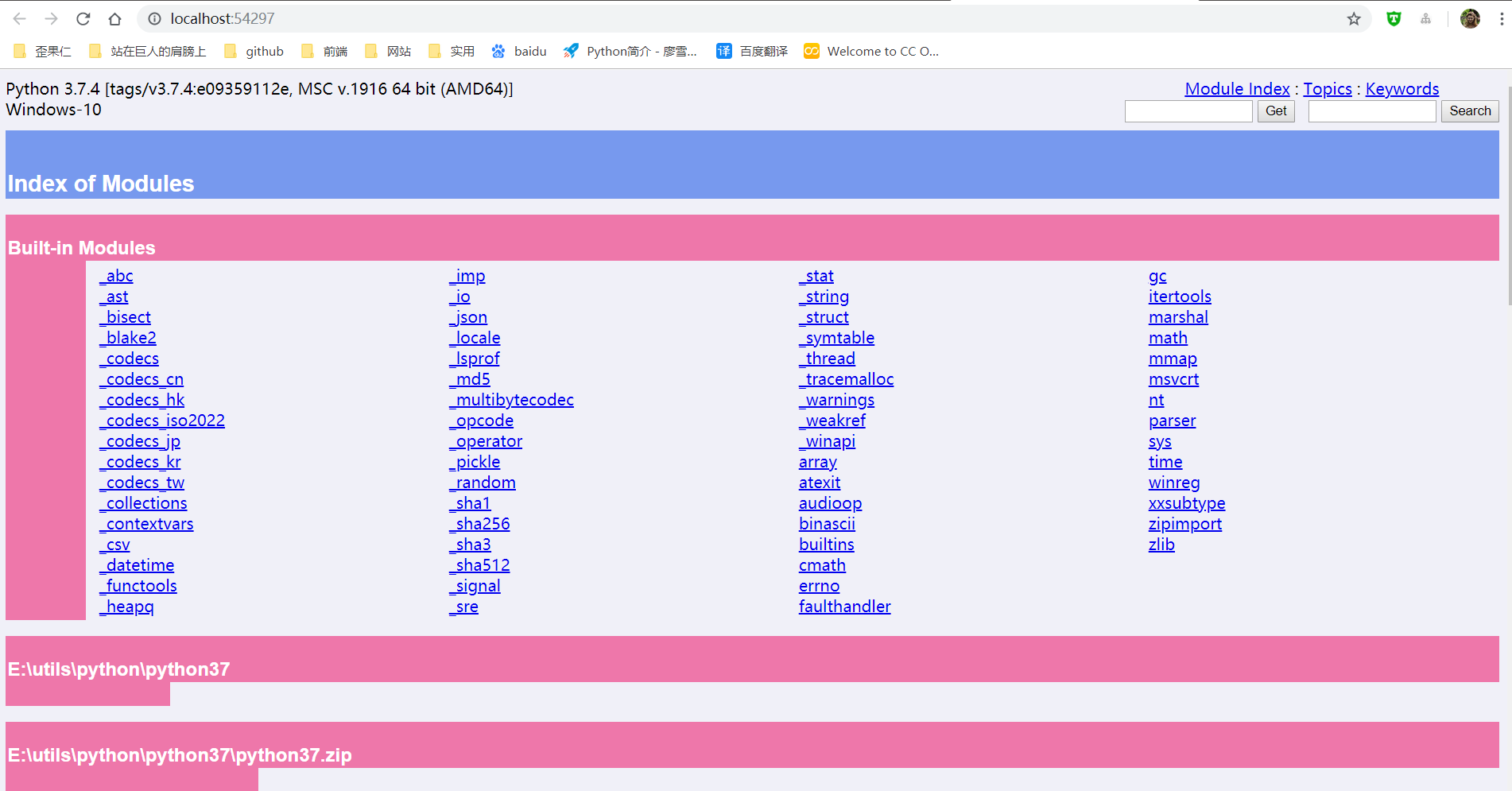IDLE（Python 3.7 64-bit） 一个Python编辑器，Python内置的集成开发工具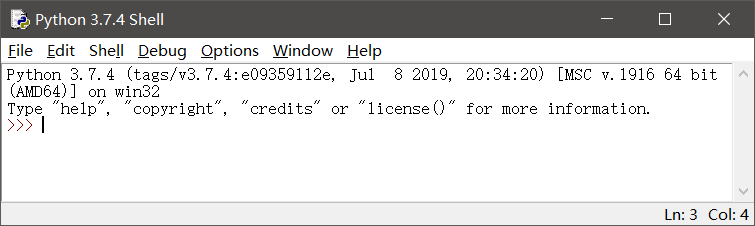Python 3.7 Manuals（64-bit） Python 3.7 开发手册  Python 3.7（64-bit） 控制台中运行Python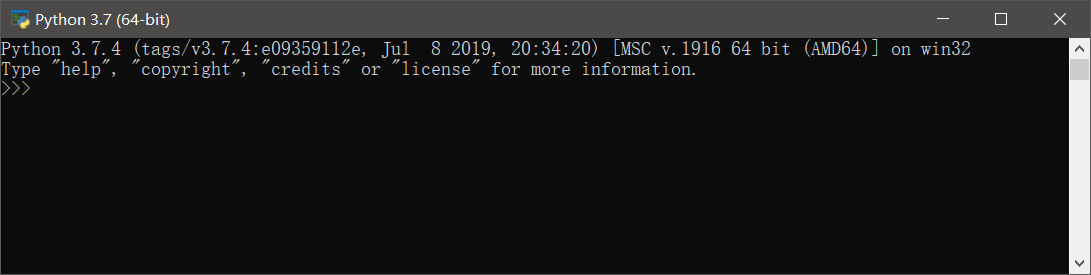二：更新pip和setuptools包，安装NumPy包，安装Matplotlib包
以下三个命令都是在控制台（windows中的cmd）中运行
更新pip和setuptools包
pip用于安装和管理Python扩展包setuptools用于发布Python包
python -m pip install -U pip setuptools

安装NumPy
Python扩展模块NumPy提供了数组和矩阵处理，以及傅立叶变换等高效的数值处理功能
 python -m pip install NumPy

安装Matplotlib包
Matplotlib是Python最著名的绘图库之一，提供了一整套和MATLAB相似的命令API，既适合交互式地进行制图，也可以作为绘图控件方便地嵌入到GUI应用程序中
python -m pip install Matplotlib

三：使用IDLE打开和执行Python源文件程序
首先： 有一个.py文件test.py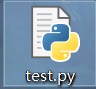使用IDLE打开.py文件的两种方式：
右键test.py---->Edit With IDLE---->Edit With IDLE 3.7（64-bit）打开IDLE，然后File---->Open（或者ctrl+O）选择.py文件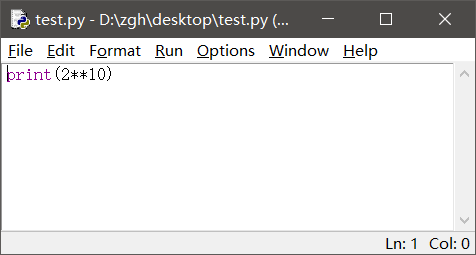运行
Run---->Run Module（或者F5） 就会出现这个界面，执行结果显示在这个界面中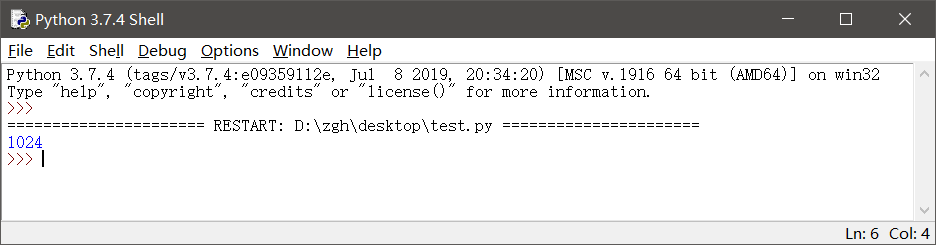补充一点： 如果在IDLE中编辑.py文件，记得修改后要保存（ctrl+s），再运行（F5）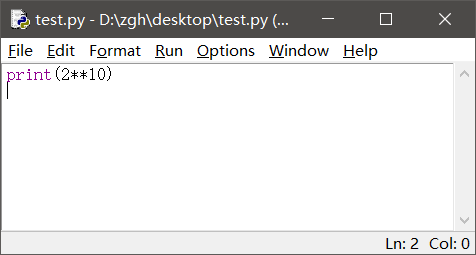四：使用资源管理器运行hello.py
hello.py文件在桌面
import random

print("hello,Python")
print("你今天的随机数字是：",random.choice(range(10)))#输出在0-9之间随机选择的整数
input()

在桌面打开PowerShell（还有两种输入方式：python hello.py或者.\hello.py）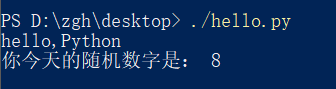或者在桌面打开cmd, 就输入hello.py或者python hello.py
补充：上述两种命令中的hello.py都是相对路径，因为文件在桌面，而且我是在桌面打开cmd，所以文件路劲可以这么简简单单的写。如果文件存储位置和cmd打开位置不一样，请使用绝对路径
五：命令行参数示例hello_argv.py
hello_argv.py文件在桌面
import sys

print("Hello,",sys.argv)
#这样写也行：
#print("Hello,"+sys.argv)

在桌面打开PowerShell（还有两种输入方式：python hello_argv.py 任意输入或者./hello_argv.py 任意输入）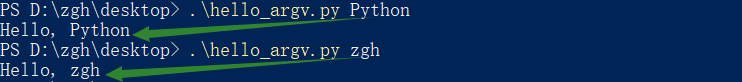或者在桌面打开cmd，就输入hello_argv.py 任意输入或者python hello_argv.py 任意输入
补充：以图中第一个命令举例，hello_argv.py即sys.argv；Python即sys.argv
第二章 Python语言基础

选择题：1、3、7、8
1. 在Python中，以下标识符合法的是
A. _B. 3CC. it’sB. str
答案：A

标识符的第一个字符必须是字母，下划线（_）；其后的字符可以是字母、下划线或数字。一些特殊的名称，作为python语言的保留关键字，不能作为标识符以双下划线开始和结束的名称通常具有特殊的含义。例如__init__为类的构造函数，一般应避免使用

B：以数字开头，错误 C：使用了'，不是字母、下划线或数字 D：str是保留关键字
3. 在下列Python语句中非法的是
A. x = y =1B. x = (y =1)C. x,y = y,xB. x=1;y=1
答案：B，C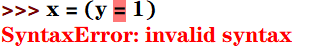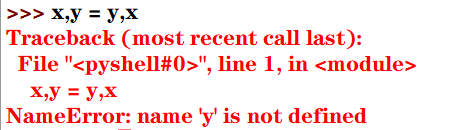7. 为了给整型变量x，y，z赋初值10，下面Python赋值语句正确的是
A. xyz=10B. x=10 y=10 z=10C. x=y=z=10B. x=10，y=10，z=10
答案：C

分号;用于在一行书写多个语句python支持链式赋值

A：赋值对象是xyz B：分号;用于在一行书写多个语句，而不是' '（即空格） D：分号;用于在一行书写多个语句，而不是,
8. 为了给整型变量x，y，z赋初值5，下面Python赋值语句正确的是
A. x=5;y=5;z=5B. xyz=5C. x,y,z=10B. x=10，y=10，z=10
答案：A

Pytho能支持序列解包赋值，但是变量的个数必须与序列的元素个数一致，否则会报错

B：赋值对象是xyz C：序列解包赋值，变量的个数必须与序列的元素个数一致，否则会报错 D：分号;用于在一行书写多个语句，而不是,
思考题：9
9.下列Python语句的输出结果是
def f():pass
print(type(f()))

结果：<class 'NoneType'>

NoneType数据类型包含唯一值None，主要用于表示空值，如没有返回值的函数的结果

上机实践：2~6
2. 编写程序，输入本金、年利率和年数，计算复利（结果保留两位小数）
money = int(input("请输入本金："))
rate = float(input("请输入年利率："))
years = int(input("请输入年数："))
amount = money*((1+rate/100)**years)
print(str.format("本金利率和为：{0:2.2f}",amount))

运行：
请输入本金：1000
请输入年利率：6.6
请输入年数：10
本金利率和为：1894.84

3. 编写程序，输入球的半径，计算球的表面积和体积（结果保留两位小数）
import math
r = float(input("请输入球的半径："))
area = 4 * math.pi * r**2
volume = 4/3*math.pi*r**3
print(str.format("球的表面积为：{0:2.2f}，体积为：{1:2.2f}",area,volume))

运行：
请输入球的半径：666
球的表面积为：5573889.08，体积为：1237403376.70

4. 编写程序，声明函数getValue(b,r,n)，根据本金b，年利率r和年数n计算最终收益v
money = int(input("请输入本金："))
rate = float(input("请输入年利率(<1)："))
years = int(input("请输入年数："))

def getValue(b,r,n):
return b*(1+r)**n

print(str.format("本金利率和为：{0:2.2f}",getValue(money,rate,years)))

运行：
请输入本金：10000
请输入年利率(<1)：0.6
请输入年数：6
本金利率和为：167772.16

5. 编写程序，求解一元二次方程x2-10x+16=0
from math import sqrt
x = (10+sqrt(10*10-4*16))/2
y = (10-sqrt(10*10-4*16))/2
print(str.format("x*x-10*x+16=0的解为：{0:2.2f}，{1:2.2f}",x,y))

运行：
x*x-10*x+16=0的解为：8.00，2.00

6. 编写程序，提示输入姓名和出生年份，输出姓名和年龄
import datetime
sName = str(input("请输入您的姓名："))
birthday = int(input("请输入您的出生年份："))
age = datetime.date.today().year - birthday
print("您好！{0}。您{1}岁。".format(sName,age))

运行：
请输入您的姓名：zgh
请输入您的出生年份：1999
您好！zgh。您20岁。

案例研究：使用Pillow库处理图像文件
https://blog.csdn.net/Zhangguohao666/article/details/102060722
通过此案例，进一步了解Python的基本概念：模块、对象、方法和函数的使用
第三章 程序流程控制

几个例题
一：编程判断某一年是否为闰年

闰年：年份能被4整除但不能被100整除，或者可以被400整除。 口诀：四年一闰，百年不闰，四百必闰

代码一：
y = int(input("请输入要判断的年份："))
if((y % 4 == 0 and y % 100 != 0) or y % 400 == 0):
print("是闰年")
else:
print("不是闰年")

代码二（使用calendar模块的isleap()函数来判断）：
from calendar import isleap

y = int(input("请输入要判断的年份："))
if(isleap(y)):print("闰年")
else:print("不是闰年")

二：利用嵌套循环打印九九乘法表
九九乘法表：
for i in range(1,10):
s = ""
for j in range(1,10):
s += str.format("%d * %d = %02d  " %(i, j, i*j))
print(s)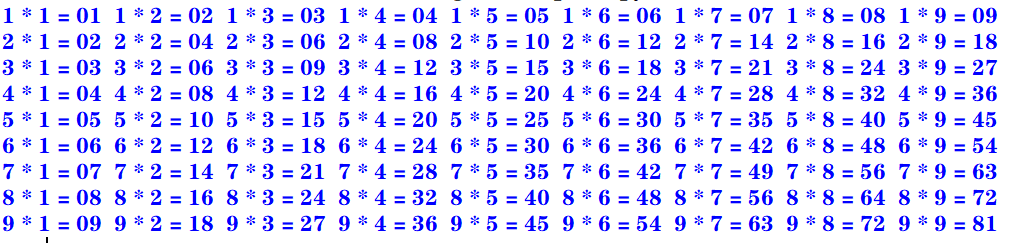下三角：
for i in range(1,10):
s = ""
for j in range(1,i+1):
s += str.format("%d * %d = %02d  " %(i, j, i*j))
print(s)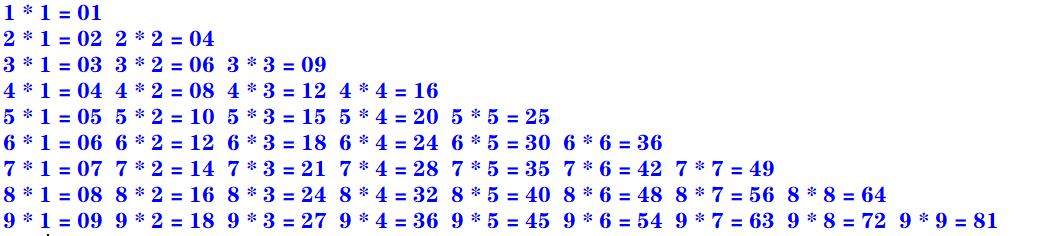上三角：
for i in range(1,10):
s = ""
for k in range(1,i):
s += "                   "
for j in range(i,10):
s += str.format("%d * %d = %02d  " %(i, j, i*j))
print(s)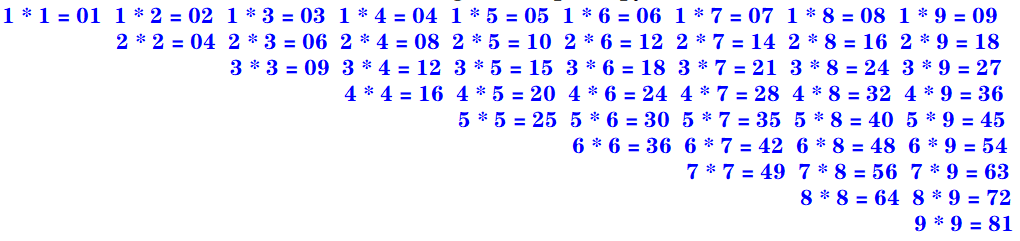三：enumerate()函数和下标元素循环示例

Python语言中的for循环直接迭代对象集合中的元素，如果需要在循环中使用索引下标访问集合元素，则可以使用内置的enumerate()函数

enumerate()函数用于将一个可遍历的数据对象（例如列表、元组或字符串）组合为一个索引序列，并返回一个可迭代对象，故在for循环当中可直接迭代下标和元素
seasons = ["Spring","Summer","Autumn","Winter"]
for i,s in enumerate(seasons,start=1):    #start默认从0开始
print("第{0}个季节：{1}".format(i,s))

运行：
第1个季节：Spring
第2个季节：Summer
第3个季节：Autumn
第4个季节：Winter

四：zip()函数和并行循环示例

如果需要并行遍历多个可迭代对象，则可以使用Python的内置函数zip()

zip()函数将多个可迭代对象中对应的元素打包成一个个元组，然后返回一个可迭代对象。如果元素的个数不一致，则返回列表的长度与最短的对象相同。
利用运算符*还可以实现将元组解压为列表
evens = [0,2,4,6,8]
odds = [1,3,5,7,9]
for e,o in zip(evens,odds):
print("{0} * {1} = {2}".format(e,o,e*o))

运行：
0 * 1 = 0
2 * 3 = 6
4 * 5 = 20
6 * 7 = 42
8 * 9 = 72

五：map()函数和循环示例

如果需要遍历可迭代对象，并使用指定函数处理对应的元素，则可以使用Python的内置函数map()

map(func,seq1[,seq2,...])

func作用于seq中的每一个元素，并将所有的调用结果作为可迭代对象返回。如果func为None，该函数的作用等同于zip()函数
计算绝对值：
>>> list(map(abs, [-1, 0, 7, -8]))
[1, 0, 7, 8]

计算乘幂：
>>> list(map(pow, range(5), range(5)))
[1, 1, 4, 27, 256]

计算ASCII码：
>>> list(map(ord, 'zgh'))
[122, 103, 104]

字符串拼接（使用了匿名函数lambda）：
>>> list(map(lambda x, y: x+y, 'zgh', '666'))
['z6', 'g6', 'h6']

选择题：1、2、3
1. 下面的Python循环体的执行次数与其他不同的是
A.
i = 0
while(i <= 10):
print(i)
i = i + 1

B.
i = 10
while(i > 0):
print(i)
i = i - 1

C.
for i in range(10):
print(i)

D.
for i in range(10,0,-1):
print(i)

答案：A
A：[0,10] 执行11次 B：[10,1] 执行10次 C：[0,9) 执行10次 D：[10,0) 执行10次
2. 执行下列Python语句将产生的结果是
x = 2; y = 2.0
if(x == y): print("Equal")
else: print("Not Equal")

A. EqualB. Not EqualC. 编译错误D. 运行时错误
答案：A

Python中的自动类型转换：
自动类型转换注意针对Number数据类型来说的当2个不同类型的数据进行运算的时候,默认向更高精度转换数据类型精度从低到高:bool int float complex关于bool类型的两个值：True 转化成整型是1；False 转化成整型是0

int类型的2转化为float类型的2.0
3. 执行下列Python语句将产生的结果是
i= 1
if(i): print(True)
else: print(False)

A. 输出1B. 输出TrueC. 输出FalseD. 编译错误
答案：B
在Python中，条件表达式最后被评价为bool值True或False。
如果表达式的结果为数值类型（0），空字符串（""），空元组（()），空列表（[]），空字典（{}），其bool值为False，否则其bool值为True
填空题：6
6. 要使语句for i in range(_,-4,-2)循环执行15次，则循环变量i的初值应当为
答案：26或者25
一开始我给的答案是26，经过评论区 亻的提醒：>>> a = 0
>>> for i in range(26, -4, -2): a+=1

>>> print(a)
15

>>> a = 0
>>> for i in range(25, -4, -2): a+=1

>>> print(a)
15

这种题目有一个规律：for i in range(x,y,z): 若循环中没有break或者continue语句， 执行次数的绝对值：result = (x-y)÷z
但实际上没有这么简单：
如果步长为 -1或者1，那么答案只有一个如果步长为 -2或者2，那么答案有两个如果步长为 -3或者3，那么答案有三个…
通过公式算出 x 之后，
如果步长为2，还要计算 (x ± 1) - z × (result-1) 的值，然后再经过琐碎的判断即可如果步长为3，还要计算 (x ± 2) - z × (result-1) 的值，……
虽然看着麻烦，但实际上是很好理解的
思考题：3~6
3. 阅读下面的Python程序，请问程序的功能是什么？
from math import sqrt

n = 0
for m in range(101,201,2):
k = int(sqrt(m))
for i in range(2, k+2):
if m % i == 0:break
if i == k + 1:
if n % 10 == 0:print()
print('%d' % m,end = " ")
n += 1

输出101到200之间的素数 每行输出10个，多余换行
运行：
101 103 107 109 113 127 131 137 139 149
151 157 163 167 173 179 181 191 193 197
199


素数（质数）是指在大于1的自然数中，除了1和它本身以外不再有其他因数的自然数。

4. 阅读下面的Python程序，请问输出的结果使什么？
n = int(input("请输入图形的行数："))
for i in range(0, n):
for j in range(0, 10 - i):print(" ", end=" ")
for k in range(0, 2 * i + 1):print(" * ", end=" ")
print("\n")

输出的是一个金字塔
运行：
请输入图形的行数：4
*

*   *   *

*   *   *   *   *

*   *   *   *   *   *   *

5. 阅读下面的Python程序，请问输出的结果使什么？程序的功能是什么？
for i in range(100,1000):
n1 = i // 100
n2 = i // 10 % 10
n3 = i % 10
if(pow(n1, 3) + pow(n2, 3) + pow(n3, 3) == i):print(i, end=" ")

输出三位数中所有的水仙花数
运行：
153 370 371 407


水仙花数 是指一个 3 位数，它的每个位上的数字的 3次幂之和等于它本身

6. 阅读下面的Python程序，请问输出的结果使什么？程序的功能是什么？
for n in range(1,1001):
total = 0; factors = []
for i in range(1, n):
if(n % i == 0):
factors.append(i)
total += i
if(total == n):print("{0} : {1}".format(n, factors))

输出1到1000的所有完数，并输出每个完数的所有因子
运行：
6 : [1, 2, 3]
28 : [1, 2, 4, 7, 14]
496 : [1, 2, 4, 8, 16, 31, 62, 124, 248]


完数 所有的真因子（即除了自身以外的约数）的和（即因子函数），恰好等于它本身

上机实践：2~14
2. 编写程序，计算1=2+3+…+100之和
使用for循环（递增）：
total = 0
for i in range(101):
total += i
print(total)

使用求和公式：
>>> (1 + 100) * 100 /2
5050.0

使用累计迭代器itertools.accumulate
>>> import itertools
>>> list(itertools.accumulate(range(1, 101)))
5050

3. 编写程序，计算10+9+8+…+1之和
使用for循环（递增）：
total = 0
for i in range(11):
total += i
print(total)

使用for循环（递减）：
total = 0
for i in range(10,0,-1):
total += i
print(total)

使用求和公式：
>>> (1 + 10) * 10 / 2
55.0

使用累计迭代器itertools.accumulate
>>> import itertools
>>> list(itertools.accumulate(range(1,11)))
55

4. 编写程序，计算1+3+5+7+…+99之和
使用for循环（递增）：
total = 0
for i in range(1,100,2):
total += i
print(total)

使用求和公式：
>>> (1 + 99) * 50 /2
2500.0

使用累计迭代器itertools.accumulate
>>> import itertools
>>> list(itertools.accumulate(range(1,100,2)))
2500

5. 编写程序，计算2+4+6+8+…+100之和
使用for循环（递增）：
total = 0
for i in range(2,101,2):
total += i
print(total)

使用求和公式：
>>> (2 + 100) * 50 / 2
2550.0

使用累计迭代器itertools.accumulate
>>> import itertools
>>> x = list(itertools.accumulate(range(2,101,2)))
>>> x[len(x)-1]
2550

6. 编写程序，使用不同的实现方法输出2000~3000的所有闰年
代码一：
for y in range(2000,3001):
if((y % 4 == 0 and y % 100 != 0) or y % 400 == 0):
print(y,end = ' ')

代码二（使用calendar模块的isleap()函数来判断）：
from calendar import isleap

for y in range(2000,3001):
if(isleap(y)):print(y,end = " ")

运行：
2000 2004 2008 2012 2016 2020 2024 2028 2032 2036 2040 2044 2048 2052 2056 2060 2064 2068 2072 2076 2080 2084 2088 2092 2096 2104 2108 2112 2116 2120 2124 2128 2132 2136 2140 2144 2148 2152 2156 2160 2164 2168 2172 2176 2180 2184 2188 2192 2196 2204 2208 2212 2216 2220 2224 2228 2232 2236 2240 2244 2248 2252 2256 2260 2264 2268 2272 2276 2280 2284 2288 2292 2296 2304 2308 2312 2316 2320 2324 2328 2332 2336 2340 2344 2348 2352 2356 2360 2364 2368 2372 2376 2380 2384 2388 2392 2396 2400 2404 2408 2412 2416 2420 2424 2428 2432 2436 2440 2444 2448 2452 2456 2460 2464 2468 2472 2476 2480 2484 2488 2492 2496 2504 2508 2512 2516 2520 2524 2528 2532 2536 2540 2544 2548 2552 2556 2560 2564 2568 2572 2576 2580 2584 2588 2592 2596 2604 2608 2612 2616 2620 2624 2628 2632 2636 2640 2644 2648 2652 2656 2660 2664 2668 2672 2676 2680 2684 2688 2692 2696 2704 2708 2712 2716 2720 2724 2728 2732 2736 2740 2744 2748 2752 2756 2760 2764 2768 2772 2776 2780 2784 2788 2792 2796 2800 2804 2808 2812 2816 2820 2824 2828 2832 2836 2840 2844 2848 2852 2856 2860 2864 2868 2872 2876 2880 2884 2888 2892 2896 2904 2908 2912 2916 2920 2924 2928 2932 2936 2940 2944 2948 2952 2956 2960 2964 2968 2972 2976 2980 2984 2988 2992 2996

7. 编写程序，计算Sn=1-3+5-7+9-11…
代码一：
n = int(input("项数："))
total = 0
flag = True
for i in range(1,2*n,2):
if(flag):
total += i
flag = False
else:
total -= i
flag = True
print(total)

代码二：
n = int(input("项数："))
total = 0
x = 2
for i in range(1,2*n,2):
total += pow(-1,x)*i
x += 1
print(total)

运行：
项数：10
-10

8. 编写程序，计算Sn=1+1/2+1/3+…
n = int(input("项数："))
total = 0.0
for i in range(1,n+1):
total += 1/i
print(total)

运行：
项数：10
2.9289682539682538

9. 编写程序，打印九九乘法表。要求输入九九乘法表的各种显示效果（上三角，下三角，矩形块等方式）
矩形块：
for i in range(1,10):
s = ""
for j in range(1,10):
s += str.format("%d * %d = %02d  " %(i, j, i*j))
print(s)下三角：
for i in range(1,10):
s = ""
for j in range(1,i+1):
s += str.format("%d * %d = %02d  " %(i, j, i*j))
print(s)上三角：
for i in range(1,10):
s = ""
for k in range(1,i):
s += "                   "
for j in range(i,10):
s += str.format("%d * %d = %02d  " %(i, j, i*j))
print(s)10. 编写程序，输入三角形的三条边，先判断是否可以构成三角形，如果可以，则进一步求三角形的周长和面积，否则报错“无法构成三角形！”
from math import sqrt

a = float(input("请输入三角形的边长a："))
b = float(input("请输入三角形的边长b："))
c = float(input("请输入三角形的边长c："))

if(a < b): a,b = b,a
if(a < c): a,c = c,a
if(b < c): b,c = c,b

if(a < 0 or b < 0 or c < 0 or b+c <= a): print("无法构成三角形！")
else:
h = (a+b+c)/2
area = sqrt(h*(h-a)*(h-b)*(h-c))
print("周长：{0}，面积：{1}".format(a+b+c,area))

运行：
请输入三角形的边长a：4
请输入三角形的边长b：3
请输入三角形的边长c：5
周长：12.0，面积：6.0

11. 编写程序，输入x，根据如下公式计算分段函数y的值。请分别用单分支语句，双分支语句结构以及条件运算语句等方法实现
y = (x2-3x)/(x+1) + 2π + sinx （x≥0 ） y = ln(-5x) + 6√(|x|+e4) - (x+1)3 (x<0)
单分支语句：
import math

x = float(input("请输入x："))
if(x >= 0):
y = (x*x - 3*x)/(x+1) + 2*math.pi + math.sin(x)
if(x < 0):
y = math.log(-5*x) + 6 * math.sqrt(abs(x) + math.exp(4)) - pow(x+1,3)

print(y)


双分支语句：
import math

x = float(input("请输入x："))
if(x >= 0):
y = (x*x - 3*x)/(x+1) + 2*math.pi + math.sin(x)
else:
y = math.log(-5*x) + 6 * math.sqrt(abs(x) + math.exp(4)) - pow(x+1,3)

print(y)

条件运算语句：
import math

x = float(input("请输入x："))
y = ((x*x - 3*x)/(x+1) + 2*math.pi + math.sin(x)) if(x >= 0) \
else (math.log(-5*x) + 6 * math.sqrt(abs(x) + math.exp(4)) - pow(x+1,3))

print(y)

运行一：
请输入x：666
668.2715406628656

运行二：
请输入x：-666
294079794.1744833

12. 编写程序，输入一元二次方程的3个系数a、b、c，求ax2+bx+c=0方程的解
import math

a = float(input("请输入系数a："))
b = float(input("请输入系数b："))
c = float(input("请输入系数c："))

delta = b*b -4*a*c

if(a == 0):
if(b == 0): print("无解")
else: print("有一个实根：",-1*c/b)
elif(delta == 0): print("有两个相等实根：x1 = x2 = ", (-1*b)/(2*a))
elif(delta > 0): print("有两个不等实根：x1 = {0}，x2 = {1}".format\
((-1*b +math.sqrt(delta))/2*a,(-1*b -math.sqrt(delta))/2*a))
elif(delta < 0): print("有两个共轭复根：x1 = {0}，x2 = {1}".format\
(complex( (-1*b)/(2*a),math.sqrt(delta*-1)/(2*a)),complex( (-1*b)/(2*a),-1*math.sqrt(delta*-1)/(2*a))))

运行一：
请输入系数a：0
请输入系数b：0
请输入系数c：10
无解

运行二：
请输入系数a：0
请输入系数b：10
请输入系数c：5
有一个实根： -0.5

运行三：
请输入系数a：1
请输入系数b：8
请输入系数c：16
有两个相等实根：x1 = x2 =  -4.0

运行四：
请输入系数a：1
请输入系数b：-5
请输入系数c：6
有两个不等实根：x1 = 3.0，x2 = 2.0

运行五：
请输入系数a：5
请输入系数b：2
请输入系数c：1
有两个共轭复根：x1 = (-0.2+0.4j)，x2 = (-0.2-0.4j)

13. 编写程序，输入整数n（n≥0）,分别利用for循环和while循环求n!
for循环
n = int(input("请输入n："))

if(n == 0): total = 1
if(n > 0):
total = 1
for i in range(n,0,-1):
total *= i

print(total)


while循环
n = int(input("请输入n："))

if(n == 0): total = 1
if(n > 0):
total = 1
while(n >= 1):
total *= n
n -= 1

print(total)

补充一个：使用累计迭代器itertools.accumulate
>>> import itertools, operator
>>> n = int(input('请输入n：'))
请输入n：7
>>> x = list(accumulate(range(1, n+1), operator.mul))
>>> x[len(x)-1]
5040

14. 编写程序，产生两个0~100（包含0和100）的随机整数a和b，求这两个整数的最大公约数和最小公倍数
现有知识点解决方法

import random

a = random.randint(0,100)
b = random.randint(0,100)
sum = a*b

print(a) #输出原来的a，b
print(b)

if(a < b): a,b = b,a

while(a%b != 0):
a,b = b,a%b

print("最大公约数：{0}，最小公倍数：{1}".format(b,sum/b))


补充：使用生成器（generate）函数：yield
>>> def func(a, b):
if(a < b): a,b = b,a
while(a%b != 0):
a,b = b,a%b
yield b

>>> import random
>>> if __name__ == '__main__':
a = random.randint(0,100)
b = random.randint(0,100)
sum = a*b
print(a,b)
t = list(iter(func(a, b)))
gcd = t[len(t)-1]
print("gcd = {0}, mcm = {1}".format(gcd, sum/gcd))

29 65
gcd = 1, mcm = 1885.0

补充：使用math模块中的gcd(x,y)函数
>>> import random
>>> import math
>>> if __name__ == '__main__':
a = random.randint(0,100)
b = random.randint(0,100)
sum = a*b
print(a,b)
gcd = math.gcd(a,b)
print("gcd = {0}, mcm = {1}".format(gcd, sum/gcd))

29 48
gcd = 1, mcm = 1392.0

案例研究：使用嵌套循环实现图像处理算法
https://blog.csdn.net/Zhangguohao666/article/details/103935185
通过图像处理算法案例，深入了解Python数据结构和基本算法流程
第四章 常用内置数据类型

几个例题
一：Python内置数据类型概述
Python中一切皆为对象，而每个对象属于某个数据类型

Python的数据类型包括：
内置的数据类型模块中定义的数据类型用户自定义的类型

四种内置的数值类型：int，float，bool，complex

int 与其他计算机语言有精度限制不同，Python中的整数位数可以为任意长度（只受限于计算机内存）。 整型对象是不可变对象。float 与其他计算机语言中的double和float对应 Python的浮点类型的精度和系统相关boolcomplex 当数值字符串中包含虚部j（或J）时即复数字面量

序列数据类型：str，tuple，bytes，list，bytearray

序列数据类型表示若干有序数据.

不可变序列数据类型：
str（字符串） 表示Unicode字符序列，例如：“zgh666” 在Python中没有独立的字符数据类型，字符即长度为1的字符串tuple（元组） 表示任意数据类型的序列，例如：(“z”,“g”,“h”,6,6,6)bytes（字节序列） 表示字节（8位）序列数据

可变序列数据类型：
list（列表） 表示可以修改的任意类型数据的序列，比如：[‘z’,‘g’,‘h’,6,6,6]bytearray（字节数组） 表示可以修改的字节（8位）数组

集合数据类型：set，frozenset

集合数据类型表示若干数据的集合，数据项目没有顺序，且不重复
set（集） 例如：{1，2，3}frozenset（不可变集）

字典数据类型：dict

字典数据类型用于表示键值对的字典 例如：{1:"zgh", 2:666}

NoneType，NotImplementedType，EllipsisType

NoneType数据类型包含唯一值None，主要用于表示空值，如没有返回值的函数的结果NotImplementedType数据类型包含唯一值NotImplemented，在进行数值运算和比较运算时，如果对象不支持，则可能返回该值EllipsisType数据类型包含唯一值Ellipsis，表示省略字符串符号...

其他数据类型

Python中一切对象都有一个数据类型，模块、类、对象、函数都属于某种数据类型 Python解释器包含内置类型， 例如： 代码对象Code objects 框架对象Frame objects 跟踪对象Traceback objects 切片对象Slice objects 静态方法对象Static method objects 类方法对象Class method objects

二：整型字面量示例

Python3.7支持使用下划线作为整数或者浮点数的千分位标记，以增强大数值的可阅读性。 二进制、八进制、十六进制则使用下划线区分4位标记

1_000_000_000  #输出1000000000

0xff_ff_ff_ff  #输出4294967295
0x_FF_FF_FF_FF  #输出4294967295

三：字符串字面量示例

两个紧邻的字符串，如果中间只有空格分隔，则自动拼接位一个字符串

'zgh' '666'  #输出'zgh666'
'zgh' + "666"   #输出'zgh666'

四：转义字符示例

转义字符后跟Unicode编码也可以表示字符
\ooo八进制Unicode码对应的字符\xhh十六进制Unicode码对应的字符

'\101'  #输出'A'
'\x41'  #输出'A'


使用r’‘或者R’'的字符串称为原始字符串，其中包含的任何字符都不进行转义

s = r'换\t行\t符\n'
s  		  #输出：'换\\t行\\t符\\n'
print(s)  #输出：换\\t行\\t符\\n

五：字符串的格式化
一：
"student number：{0}，score_average：{1}".format(2,100)
#输出：'student number：2，score_average：100'

二：
str.format("student number：{0}，score_average：{1}",2,100)
#输出：'student number：2，score_average：100'

三（兼容Python2的格式，不推荐使用）：
 "student number：%4d，score_average：%2.1f" %(2,100)
#输出：'student number：   2，score_average：100.0'

六：字符串示例，格式化输出字符串堆积的三角形

str.center()方法用于字符串两边填充str.rjust()方法用于字符串右填充

print("1".center(20))		#一行20个字符，居中对齐
print(format("121","^20"))	#一行20个字符，居中对齐
print("1".rjust(20,"*"))	#一行20个字符，右对齐，加*
print(format("121","*>20"))	#一行20个字符，右对齐，加*

运行：
         1
121
*******************1
*****************121

选择题：11
11. 关于Python字符串，下列说法错误的是
A. 字符即长度为1的字符串 B. 字符串以/0标识字符串的结束 C. 用户既可以用单引号，也可以用双引号创建字符串 D. 用三引号字符串中可以包含换行回车等特殊字符
答案：B

Python中字符串不是用\0来判断字符串结尾， 每个字符串都存有字符串的长度，通过计数来判断是否到达结尾。

虽然在c语言中\0就是来判断字符串的结尾;
填空题：4、7、8、9、10、13、21
4. Python表达式3 ** 2 ** 3的值为
答案：6561

表达式中，相同优先级的运算，从右往左

7. Python语句print(pow(-3,2),round(18.67,1),round(18.67,-1))的输出结果是
答案：9 18.7 20.0

pow()幂运算 round()四舍六入，五留双

8. Python语句print(round(123.84,0),round(123.84,-2),floor(15.5))的输出结果是
答案：124.0 100.0 15

补充：floor()是math模块中的方法，向下取整

9. Python语句print(int(‘20’,16),int(‘101’,2))的输出结果是
答案：32 5

注意：int(x,y)是指将y进制的数值x转化为10进制数

10. Python语句print(hex(16),bin(10))的输出结果是
答案：0x10 0b1010

hex(x)将十进制数x转化为十六进制，以字符串形式输出 bin(x)将十进制数x转化为二进制，以字符串形式输出

13. Python语句print(gcd(12,16),divmod(7,3))的输出结果是
答案：4 (2,1)

gcd()是math模块中的函数，求最大公约数 divmod()是内置函数，返回商和余数

21. Python语句序列 x=True;y=False;z=False;print(x or y and z) 的运行结果是
答案：True

and优先级比or高

思考题：5
5. 阅读下面的Python程序，请问输出结果是什么？
from decimal import *

ctx = getcontext()
ctx.prec = 2
print(Decimal('1.78'))#1.78
print(Decimal('1.78') + 0)#1.8
ctx.rounding = ROUND_UP
print(Decimal('1.65') + 0)#1.7
print(Decimal('1.62') + 0)#1.7
print(Decimal('-1.45') + 0)#-1.5
print(Decimal('-1.42') + 0)#-1.5
ctx.rounding = ROUND_HALF_UP
print(Decimal('1.65') + 0)#1.7
print(Decimal('1.62') + 0)#1.6
print(Decimal('-1.45') + 0)#-1.5
ctx.rounding = ROUND_HALF_DOWN
print(Decimal('1.65') + 0)#1.6
print(Decimal('-1.45') + 0)#-1.4

上机实践：2~14
2. 编写程序，格式化输出杨辉三角

杨辉三角即二项式定理的系数表，各元素满足如下条件：第一列及对角线上的元素均为1；其余每个元素等于它上一行同一列元素与前一列元素之和

我使用了一个更加精妙的规律 比如第一行为1 第二行：01 + 10 = 11 第三行：011 + 110 = 121 第四行：0121 + 1210 = 1331 。。。
def generate(numRows):
l1 = 
n = 0
while n < numRows:
print(str(l1).center(66))
l1 = [sum(t) for t in zip( + l1, l1 + )]  #利用zip函数算出每一行 如第二行 zip([0,1],[1,0])=[1,1],以此类推
n += 1
a=int(input("请输入行数"))
generate(a)

运行：
请输入行数4

[1, 1]
[1, 2, 1]
[1, 3, 3, 1]

3. 输入直角三角形的两个直角边，求三角形的周长和面积，以及两个锐角的度数。结果保留一位小数
import math

a = float(input("请输入直角三角形的直角边a："))
b = float(input("请输入直角三角形的直角边b："))
c = math.sqrt(a*a+b*b)

p = a + b + c
area = 0.5*a*b
print("三角形的周长：{0:1.1f}，面积：{1:1.1f}".format(p,area))

sina = a/c
sinb = b/c

a_degree = round(math.asin(sina) * 180 / math.pi,0)
b_degree = round(math.asin(sinb) * 180 / math.pi,0)

print("三角形直角边a的度数：{0}，b的度数：{1}".format(a_degree,b_degree))

运行：
请输入直角三角形的直角边a：3
请输入直角三角形的直角边b：4
三角形的周长：12.0，面积：6.0
三角形直角边a的度数：37.0，b的度数：53.0

4. 编程产生0~100（包含0和100）的三个随机数a、b、c，要求至少使用两种不同的方法，将三个数按从小到大的顺序排序
方法一：
import random

a = random.randint(0, 100)
b = random.randint(0, 100)
c = random.randint(0, 100)

print(str.format("原始值：{0}，{1}，{2}", a, b, c))

if(a > b): a,b = b,a
if(a > c): a,c = c,a
if(b > c): b,c = c,b

print(str.format("增序：{0}，{1}，{2}", a, b, c))

方法二（使用内置函数max、min、sum）：
import random

a = random.randint(0, 100)
b = random.randint(0, 100)
c = random.randint(0, 100)

print(str.format("原始值：{0}，{1}，{2}", a, b, c))

maxx = max(a, b, c)
minx = min(a, b, c)
median = sum([a, b, c]) - minx - maxx

print(str.format("增序：{0}，{1}，{2}", minx, median, maxx))

方法三（使用内置函数sorted）：
>>> import random
>>> a = random.randint(0,100)
>>> b = random.randint(0,100)
>>> c = random.randint(0,100)
>>> print("init value: {0} , {1} , {2}".format(a,b,c))
init value: 17 , 6 , 59
>>> sorted([a,b,c])
[6, 17, 59]

5. 编程计算有固定工资收入的党员每月所缴纳的党费。
工资基数3000元及以下者，交纳工资基数的0.5% 工资基数3000~5000元者，交纳工资基数的1% 工资基数在5000~10000元者，交纳工资基数的1.5% 工资基数超过10000元者，交纳工资基数的2%
salary = float(input("请输入有固定工资收入的党员的月工资："))
if salary <= 3000: dues = salary*0.005
elif salary <= 5000: dues = salary*0.01
elif salary <= 10000: dues = salary*0.15
else: dues = salary*0.02

print("交纳党费：",dues)

运行：
请输入有固定工资收入的党员的月工资：10001
交纳党费： 200.02

6. 编程实现袖珍计算器，要求输入两个操作数和一个操作符（+、-、*、/、%），根据操作符输出运算结果。注意/和%运算符的零异常问题
a = float(input("请输入操作数（左）："))
b = float(input("请输入操作数（右）："))
operator = input("请输入操作符（+、-、*、/、%）：")

if(b == 0 and (operator == '/' or operator == '%')):
print("分母为零，异常！")
else:
if operator == '+': result = a+b
elif operator == '-': result = a-b
elif operator == '*': result = a*b
elif operator == '/': result = a/b
elif operator == '%': result = a%b
print("{0} {1} {2}= {3}：".format(a,operator,b,result))

运行：
请输入操作数（左）：10
请输入操作数（右）：5
请输入操作符（+、-、*、/、%）：+
10.0 + 5.0= 15.0：

7. 输入三角形的3条边a、b、c，判断此3边是否可以构成三角形。若能，进一步判断三角形的性质，即为等边、等腰、直角或其他三角形
a = float(input("请输入三角形的边a："))
b = float(input("请输入三角形的边b："))
c = float(input("请输入三角形的边c："))

if(a > b): a,b = b,a
if(a > c): a,c = c,a
if(b > c): b,c = c,b

result = "三角形"
if(not(a>0 and b>0 and c>0 and a+b>c)):
result = '此三边无法构成三角形'
else:
if a == b == c: result = '等边三角形'
elif(a==b or a==c or b==c): result = '等腰三角形'
elif(a*a+b*b == c*c): result = '直角三角形'

print(result)

运行：
请输入三角形的边a：3
请输入三角形的边b：4
请输入三角形的边c：5
直角三角形

8. 编程实现鸡兔同笼问题
已知在同一个笼子里共有h只鸡和兔，鸡和兔的总脚数为f，其中h和f由用户输入，求鸡和兔各有多少只？要求使用两种方法：一是求解方程；二是利用循环进行枚举测试
h = int(input("请输入总头数："))
f = int(input("请输入总脚数："))

def fun1(h,f):
rabbits = f/2-h
chicken = h-rabbits
if(chicken < 0 or rabbits < 0): return '无解'
return chicken,rabbits

def fun2(h,f):
for i in range(0,h+1):
if(2*i + 4*(h-i) == f):return i,h-i
return '无解'

if(h>0 and f>0 and f % 2 == 0):
if fun1(h,f)=='无解':
print("无解")
else:
print("方法一：鸡：{0}，兔：{1}".format(fun1(h,f),fun1(h,f)))
print("方法二：鸡：{0}，兔：{1}".format(fun2(h,f),fun2(h,f)))
else:
print('输入的数据无意义')

运行：
请输入总头数：100
请输入总脚数：100
无解

9. 输入任意实数x，计算ex的近似值，直到最后一项的绝对值小于10-6为止
ex = 1 + x + x2/2 + x3/3! + x4/4! + … + xn/n!
x = int(input("请输入任意实数："))

e = 1
i = 1
t = 1
a = 1
while(a >= 10e-6):
t *= i
a = pow(x,i)/t
e += a
i += 1

print(e)

运行：
请输入任意实数：1
2.7182815255731922

我发现了在Python中10e-6和pow(10,-6)是有差别的，将上述代码中的10e-6改为pow(10,-6)，输出结果会有细微的差别
运行：
请输入任意实数：1
2.7182818011463845

10. 输入任意实数a（a>=0），用迭代法求x=√a，要求计算的相对偏差小于10-6
求平方根的公式：
Xn+1 = 0.5(Xn + a/Xn)
import math

a = int(input("请输入任意实数a(>=0)："))

x = a / 2
y = (x + a/x) / 2

while(abs(y-x) >= pow(10,-6)):
x = y
y = (x + a/x) / 2

print(y)

运行：
请输入任意实数a(>=0)：2
1.414213562373095

11. 即有一个数，用3除余2，用5除余3，用7除余2，请问0~1000中这样的数有哪些？
我国古代有位大将，名叫韩信。他每次集合部队，只要求部下先后按1-3，1-5，1-7报数，然后再报告一下各队每次报数的余数，他就知道到了多少人。他的这种巧妙算法被人们称作“鬼谷算”，也叫“隔墙算”，或称为“韩信点兵”，外国人还称它为“中国余数定理”。
for i in range(0,1001):
if((i % 3 == 2 )and (i % 5 == 3) and (i % 7 == 2)): print(i, end="  ")

运行：
23  128  233  338  443  548  653  758  863  968

12. 一球从100米的高度自由下落，每次落地后反弹回原高度的一半，再落下。求小球在第10次落地时共经过多少米?第10次反弹多高
规律： 第一次下落时的高度：100 第二次下落时的高度（第一次反弹的高度）：50 第三次下落时的高度（第二次反弹的高度）：25 …
n = 10

h_down = 100
h_up = 0
sum = 0
for i in range(1,n+1):
sum += h_down+h_up
h_down = h_up = h_down/2

print("小球在第十次落地时共经过：{0}米，第十次反弹高度：{1}米".format(sum,h_up))

运行：
小球在第十次落地时共经过：299.609375米，第十次反弹高度：0.09765625米

13. 猴子吃桃问题
猴子第一天摘下若干个桃子，当天吃掉一半多一个；第二天接着吃了剩下的桃子的一半多一个；以后每天都吃了前一天剩下的桃子的一半多一个。到第八天发现只剩一个桃子了。请问猴子第一天共摘了多少个桃子？
这是一个递推问题
某天所剩桃子数x 后一天所剩桃子数y = x - (x/2+1) = x/2-1
则x = 2(y+1)
result = 1
for i in range(8,0,-1):
print("第{0}天桃子数：{1}".format(i,result))
result = 2*(result+1)

运行：
第8天桃子数：1
第7天桃子数：4
第6天桃子数：10
第5天桃子数：22
第4天桃子数：46
第3天桃子数：94
第2天桃子数：190
第1天桃子数：382

14. 计算Sn = 1+11+111+…+111…111（最后一项是n个1）。n是一个随机产生的1~10（包括1和10）中的正整数
import random

n = random.randint(1,10)

x = 1
s = 0
for i in range(1,n+1):
s += x
x = 10*x+1

print("n = {0}，sn = {1}".format(n,s))

运行：
n = 6，sn = 123456

random.randint(a, b)
生成指定范围内的整数范围：[a, b]
案例研究：科学计算和数据分析
https://blog.csdn.net/Zhangguohao666/article/details/103941448
通过Python科学计算和数据分析库的安装和基本使用，了解使用Python进行科学计算的基本方法
第五章 序列数据类型

几个例题
一：Python中内置的序列数据类型

元组也称为定值表，用于存储固定不变的表列表也称为表，用于存储其值可变的表字符串是包括若干字符的序列数据，支持序列数据的基本操作字节序列数据是包括若干字节的序列。Python抓取网页时返回的页面通常为utf-8编码的字节序列。

字节序列和字符串可以直接相互转换（字节编码和解码）：
>>> s1 = b'abc'
>>> s1
b'abc'
>>> s1.decode("utf-8")
abc

>>> s2 = "中国"
>>> s2.encode("utf-8")

二：序列的切片操作示例
>>> s = 'zgh666'
>>> s
'z'
>>> s
'h'
>>> s[:3]
'zgh'
>>> s[1:3]
'gh'
>>> s[3:6]
'666'
>>> s[3:55]
'666'
>>> s[::-1]
'666hgz'
>>> s[3:2]
''
>>> s[:]
'zgh666'
>>> s[::2]
'zh6'

三：序列的连接和重复操作

通过连接操作符+可以连接两个序列，形成一个新的序列对象通过重复操作符*可以重复一个序列n次连接操作符和重复操作符也支持复合赋值运算，即：+=，*=

>>> x = 'zgh'
>>> y = '666'
>>> x + y
'zgh666'
>>> x *2
'zghzgh'
>>> x += y
>>> x
'zgh666'
>>> y *= 3
>>> y
'666666666'

四：序列的成员关系操作
innot ins.count(x) x在s中出现的次数s.index(x) x在s中第一次出现的下标
>>> s = "zgh666"
>>> 'z' in s
True
>>> 'g' not in s
False
>>> s.count('6')
3
>>> s.index('6')
3

五：序列的排序操作
sorted(iterable,key=None,reverse=False)
>>> sorted(s)
[1, 3, 5, 9]
>>> sorted(s,reverse=True)
[9, 5, 3, 1]

>>> s = 'zGhZgH'
>>> sorted(s)
['G', 'H', 'Z', 'g', 'h', 'z']
>>> sorted(s,key=str.lower)
['G', 'g', 'h', 'H', 'z', 'Z']
>>> sorted(s,key=str.lower,reverse=True)
['z', 'Z', 'h', 'H', 'G', 'g']

六：序列的拆分
变量个数与序列长度相等 若变量个数与序列的元素个数不一致，将导致ValueError
>>> data = (118,'zgh',(100,100,100))
>>> sid,name,(chinese,english,math) = data
>>> sid
118
>>> name
'zgh'
>>> chinese
100
>>> english
100
>>> math
100

变量个数与序列长度不等 如果序列长度未知，可以使用*元组变量，将多个值作为元组赋值给元组变量。在一个赋值语句中，*元组变量只允许出现一次，否则将导致SyntaxError
>>> first,second,third,*middles,last = range(10)
>>> first
0
>>> second
1
>>> third
2
>>> middles
[3, 4, 5, 6, 7, 8]
>>> last
9

>>> first,*middles,last = sorted([58,60,60,100,70,70])
>>> sum(middles)/len(middles)
65.0

使用临时变量_ 如果只需要部分数据，序列的其它位置可以使用临时变量_
>>> record = ['zgh','858990471@qq.com','17354364147','15272502101']
>>> name,_,*phone = record
>>> name
'zgh'
>>> phone
['17354364147', '15272502101']

七：使用元组字面量，tuple创建元组实例对象的实例
>>> t1 = 1,2,3
>>> t1
(1, 2, 3)

>>> t2 = (4,5,6)
>>> t2
(4, 5, 6)

>>> t3 = (9,)
>>> t3
(9,)

如果元组中只有一个项目，后面的逗号不能省略。

Python解释器把(1)解释为整数1，将(1,)解释为元组

>>> t1 = tuple()
>>> t1
()

>>> t2 = tuple("zgh666")
>>> t2
('z', 'g', 'h', '6', '6', '6')

>>> t3 = tuple(['z','g','h'])
>>> t3
('z', 'g', 'h')

八：使用列表字面量，list创建列表实例对象的实例
>>> l1 = []
>>> l1
[]

>>> l2 = ['zgh666']
>>> l2
['zgh666']

>>> l3 = [(1,2,3)]
>>> l3
[(1, 2, 3)]

>>> l1 = list()
>>> l1
[]

>>> l2 = list(b'zgh666')
>>> l2
[122, 103, 104, 54, 54, 54]

>>> l3 = list(b'aAbBcC')
>>> l3
[97, 65, 98, 66, 99, 67]

补充：列表是可变对象，故用户可以改变列表对象中元素的值，也可以通过del删除某元素
九：列表解析表达式示例
使用列表解析表达式可以简单，高效地处理一个可迭代对象，并生成结果列表
>>> [(i,i**2) for i in range(10)]
[(0, 0), (1, 1), (2, 4), (3, 9), (4, 16), (5, 25), (6, 36), (7, 49), (8, 64), (9, 81)]

>>> [i for i in range(10) if i%2==0]
[0, 2, 4, 6, 8]

>>> [(x,y,x*y) for x in range(1,4) for y in range(1,4) if x>=y]
[(1, 1, 1), (2, 1, 2), (2, 2, 4), (3, 1, 3), (3, 2, 6), (3, 3, 9)]

选择题：4、5、7、11、12
4. Python语句序列“a = (1,2,3,None,(),[]);print(len(a))”的运行结果是
>>> a = (1,2,3,None,(),[])
>>> len(a)
6

5. Python语句序列“nums = set([1,2,2,3,3,3,4]);print(len(nums))”的运行结果是
>>> nums = set([1,2,2,3,3,3,4])
>>> nums
{1, 2, 3, 4}
>>> len(nums)
4

7. Python语句序列“s1=[4,5,6];s2=s1;s1=0;print(s2)”的运行结果是
Python中变量（如s1，s2）存储在栈中，存放的是地址 [4,5,6]存储在堆中
s1 = [4,5,6]即s1存储指向堆中[4,5,6]的地址 s2 = s1地址赋值，即s2和s1都指向同一个地址 所以对列表进行修改，两者的显示都会发生变化
>>> s1 = [4,5,6]
>>> s2 = s1
>>> s1 = 0
>>> s1
[4, 0, 6]
>>> s2
[4, 0, 6]

11. Python语句序列“s={‘a’,1,‘b’,2};print(s[‘b’])”的运行结果是
A. 语法错B. ‘b’C. 1D. 2
答案：A
通过值访问集合是没有意义的，语法也不支持
>>> s ={'a',1,'b',2}
>>> print(s['b'])
Traceback (most recent call last):
File "<pyshell#29>", line 1, in <module>
print(s['b'])
TypeError: 'set' object is not subscriptable

补充：集合set是无序不重复的，是无法通过下标访问的
12. Python语句print(r"\nGood")的运行结果是
A. 新行和字符串GoodB. r"\nGood"C. \nGoodD. 字符r、新行和字符串Good
答案：C
>>> print(r"\nGood")
\nGood


r""声明原始字符串

填空题：1、5、6、12、13、14
1. Python语句序列“fruits = [‘apple’,‘banana’,‘bear’];print(fruits[-1][-1])”的运行结果是
注意：fruit[-1]是字符串’bear’ 所以：fruit[-1][-1]即'bear[-1]'
>>> fruits = ['apple','banana','pear']
>>> fruits[-1]
'pear'
>>> fruits[-1][-1]
'r'

5. Python语句 print(’%d%%%d’%(3/2,3%2)) 的运行结果是
>>> print('%d%%%d'%(3/2,3%2))
1%1

6. Python语句序列“s = [1,2,3,4];s.append([5,6]);print(len(s))”的运行结果是
答案：5
注意append()和extend()函数的区别 s.append(x)将对象x追加到s尾部 s.extend(x)将序列x追加到s尾部
append
>>> s = [1,2,3,4]
>>> s.append([5,6])
>>> s
[1, 2, 3, 4, [5, 6]]
>>> len(s)
5

extend
>>> s = [1,2,3,4]
>>> s.extend([5,6])
>>> s
[1, 2, 3, 4, 5, 6]
>>> len(s)
6

12
>>> s =('a','b','c','d','e')
>>> s
'c'
>>> s[2:3]
('c',)
>>> s[2:4]
('c', 'd')
>>> s[1::2]
('b', 'd')
>>> s[-2]
'd'
>>> s[::-1]
('e', 'd', 'c', 'b', 'a')
>>> s[-2:-1]
('d',)
>>> s[-99:-5]
()
>>> s[-99:-3]
('a', 'b')
>>> s[::]
('a', 'b', 'c', 'd', 'e')
>>> s[1:-1]
('b', 'c', 'd')

13
>>> s = [1,2,3,4,5,6]
>>> s[:1] = []
>>> s
[2, 3, 4, 5, 6]

>>> s[:2] = 'a'
>>> s
['a', 4, 5, 6]

>>> s[2:] = 'b'
>>> s
['a', 4, 'b']

>>> s[2:3] = ['x','y']
>>> s
['a', 4, 'x', 'y']

>>> del s[:1]
>>> s
[4, 'x', 'y']

14
>>> s = ['a','b']
>>> s.append([1,2])
>>> s
['a', 'b', [1, 2]]
>>> s.extend('34')
>>> s
['a', 'b', [1, 2], '3', '4']
>>> s.extend([5,6])
>>> s
['a', 'b', [1, 2], '3', '4', 5, 6]
>>> s.insert(1,7)
>>> s
['a', 7, 'b', [1, 2], '3', '4', 5, 6]
>>> s.insert(10,8)
>>> s
['a', 7, 'b', [1, 2], '3', '4', 5, 6, 8]
>>> s
['a', 7, 'b', [1, 2], '3', '4', 5, 6]
>>> s.remove('b')
>>> s
['a', 7, [1, 2], '3', '4', 5, 6]
>>> s[3:] =[]
>>> s
['a', 7, [1, 2]]
>>> s.reverse()
>>> s
[[1, 2], 7, 'a']
>>>

思考题：2、3、5
2. 阅读下面的Python语句，请问输出结果是什么？
n = int(input('请输入图形的行数：'))

for i in range(n,0,-1):
print(" ".rjust(20-i),end=' ')
for j in range(2*i-1):print(" * ",end=' ')
print('\n')

for i in range(1,n):
print(" ".rjust(19-i),end=' ')
for j in range(2*i+1):print(" * ",end=' ')
print('\n')

运行一：
请输入图形的行数：1
*

运行二：
请输入图形的行数：2
*   *   *

*

*   *   *

运行三：
请输入图形的行数：3
*   *   *   *   *

*   *   *

*

*   *   *

*   *   *   *   *

3. 阅读下面的Python语句，请问输出结果是什么？
n = int(input('请输入上（或下）三角行数：'))

for i in range(0,n):
print(" ".rjust(19-i),end=' ')
for j in range(2*i+1):print(" * ",end=' ')
print('\n')

for i in range(n-1,0,-1):
print(" ".rjust(20-i),end=' ')
for j in range(2*i-1):print(" * ",end=' ')
print('\n')

运行：
请输入上（或下）三角行数：4
*

*   *   *

*   *   *   *   *

*   *   *   *   *   *   *

*   *   *   *   *

*   *   *

*

5. 阅读下面的Python语句，请问输出结果是什么？
先看这三句：
>>> names1 = ['Amy','Bob','Charlie','Daling']
>>> names2 = names1
>>> names3 = names1[:]

毫无疑问，此时names1,names2,names3的值都是[‘Amy’,‘Bob’,‘Charlie’,‘Daling’] 但是
>>> id(names1)
2338529391368
>>> id(names2)
2338529391368
>>> id(names3)
2338529391560

names1和names2指向同一个地址 而names3指向另一个地址
然后：
>>> names2 = 'Alice'
>>> names3 = 'Ben'
>>> names1
['Alice', 'Bob', 'Charlie', 'Daling']
>>> names2
['Alice', 'Bob', 'Charlie', 'Daling']
>>> names3
['Amy', 'Ben', 'Charlie', 'Daling']

最后：
>>> sum = 0
>>> for ls in(names1,names2,names3):
if ls == 'Alice': sum+=1
if ls == 'Ben':sum+=2

>>> print(sum)
4

上机实践：2~6
2. 统计所输入字符串中单词的个数，单词之间用空格分隔
s = input("请输入字符串：")

num = 0
for i in s:
if((i >= 'a' and i <= 'z') or (i >= 'A' and i <= 'Z')):
num += 1

print("其中的单词总数：",num)

运行：
请输入字符串：zgh666 ZGH6
其中的单词总数： 6

3. 编写程序，删除一个list里面重复元素
方法一：利用set集合不重复的性质（但结果不能保证原来的顺序）
l = [1,2,2,3,3,3,4,5,6,6,6]
s = set(l)
l = list(s)
print(l)

运行：
[1, 2, 3, 4, 5, 6]

方法二：既可以去除重复项，又可以保证原来的顺序
def unique(items):
items_existed = set()
for item in items:
if item not in items_existed:
yield item

if __name__ == '__main__':
a = [1, 8, 5, 1, 9, 2, 1, 10]
a1 = unique(a)
print(list(a1))


运行结果：
[1, 8, 5, 9, 2, 10]

对代码的分析：
可以看出，unique()函数返回的并不是items_existed，而是利用了yield

在函数定义中，如果使用yield语句代替return返回一个值，则定义了一个生成器函数（generator） 生成器函数是一个迭代器，是可迭代对象，支持迭代

a1 = unique(a) 这个函数返回的实际上是一个可迭代对象 若print(a1)得到的会是：<generator object unique at 0x0000016E23AF4F48>所以，要得到去掉重复后的列表的样子，需要将可迭代对象a1放在list()中 运行：
4. 编写程序，求列表[9,7,8,3,2,1,55,6]中的元素个数、最大值、最小值，以及元素之和、平均值。请思考有几种实现方法？
内置函数：
s = [9,7,8,3,2,1,55,6]

print("元素个数：{0}，最大值：{1}，最小值：{2}，和：{3}，平均值：{4}".\
format(len(s),max(s),min(s),sum(s),sum(s)/len(s)))

直接访问元素列表（for i in s…）：
s = [9,7,8,3,2,1,55,6]

sum = 0
max = s
min = s
length = 0
for i in s:
sum += i
length += 1
if(i > max): max = i
if(i < min): min = i

print("元素个数：{0}，最大值：{1}，最小值：{2}，和：{3}，平均值：{4}".\
format(length,max,min,sum,sum/length))


间接访问列表元素（for i in range(0,len(s))…）：
s = [9,7,8,3,2,1,55,6]

sum = 0
max = s
min = s
length = len(s)
for i in range(0,length):
sum += s[i]
if(s[i] > max): max = s[i]
if(s[i] < min): min = s[i]

print("元素个数：{0}，最大值：{1}，最小值：{2}，和：{3}，平均值：{4}".\
format(length,max,min,sum,sum/length))


正序访问（i=0;while i<len(s)…）：
s = [9,7,8,3,2,1,55,6]

sum = 0
max = s
min = s
length = len(s)

i = 0
while(i < length):
sum += s[i]
if(s[i] > max): max = s[i]
if(s[i] < min): min = s[i]
i += 1

print("元素个数：{0}，最大值：{1}，最小值：{2}，和：{3}，平均值：{4}".\
format(length,max,min,sum,sum/length))


反序访问（i=len(s)-1;while i>=0…）：
s = [9,7,8,3,2,1,55,6]

sum = 0
max = s
min = s
length = len(s)

i = length-1
while(i >= 0):
sum += s[i]
if(s[i] > max): max = s[i]
if(s[i] < min): min = s[i]
i -= 1

print("元素个数：{0}，最大值：{1}，最小值：{2}，和：{3}，平均值：{4}".\
format(length,max,min,sum,sum/length))


while True：…break
s = [9,7,8,3,2,1,55,6]

sum = 0
max = s
min = s
length = len(s)

i = 0
while(True):
if(i > length-1): break
sum += s[i]
if(s[i] > max): max = s[i]
if(s[i] < min): min = s[i]
i += 1

print("元素个数：{0}，最大值：{1}，最小值：{2}，和：{3}，平均值：{4}".\
format(length,max,min,sum,sum/length))

运行：
元素个数：8，最大值：55，最小值：1，和：91，平均值：11.375

5. 编写程序，将列表[9,7,8,3,2,1,5,6]中的偶数变成它的平方，奇数保持不变
l = [9,7,8,3,2,1,5,6]

for i,value in enumerate(l):
if(value % 2 == 0):l[i] = value**2

print(l)

运行：
[9, 7, 64, 3, 4, 1, 5, 36]

6. 编写程序，输入字符串，将其每个字符的ASCII码形成列表并输出
s = input("请输入一个字符串：")
l = list()
for i in s:
l.append(ord(i))

print(l)

运行：
请输入一个字符串：zgh666
[122, 103, 104, 54, 54, 54]

案例研究：猜单词游戏
https://blog.csdn.net/Zhangguohao666/article/details/103948234
通过猜单词游戏的设计和实现，帮助读者了解使用Python系列数据类型和控制流程
第六章 输入和输出

几个例题
一：运行时提示输入密码

输入密码时，一般需要不明显，则可以使用模块getpass，以保证用户输入的密码在控制台中不回显

import getpass

else:
print('failed!')

input()#为了看到输出结果。因为执行完毕后，控制台会立马关闭


注意：上面这个代码，如果使用IDLE执行，会因为安全问题而执行失败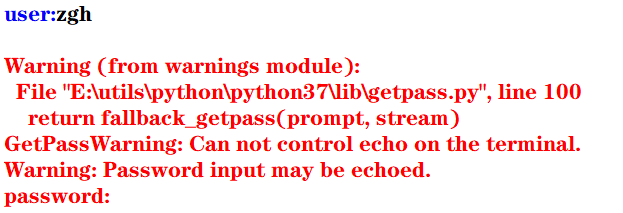但是，在控制台中执行就没问题，看输出结果（可以看到，输入的密码不会显示出来）：
user:zgh

二：重定向标准输出到一个文件的示例
这种重定向由控制台完成，而与Python本身无关。

格式： 程序 > 输出文件

其目的是将显示屏从标准输出中分离，并将输出文件与标准输出关联，即程序的执行结果将写入输出文件，而不是发送到显示屏中显示

首先准备一个test.py文件（代码如下）
import sys,random

n = int(sys.argv)
for i in range(n):
print(random.randrange(0,100))

然后在PowerShell中：python test.py 100 > scores.txt 记住，切记，一定要注意：千万能省略python，这样写./test.py 100 > scores.txt会出现问题，生成的scores文件中会没有任何内容！！！（原因未知）
然后在当前目录下，100个[0,100)范围内的的整数生成在scores.txt文件中了
三：重定向文件到标准输入

格式： 程序 < 输入文件

其目的是将控制台键盘从标准输入中分离，并将输入文件与标准输入关联，即程序从输入文件中读取输入数据，而不是从键盘中读取输入数据

准备一个average.py文件（代码如下）
import sys

total =0.0
count = 0
for line in sys.stdin:
count += 1
total += float(line)

avg = total/count
print("average:",avg)

然后问题总是不期而至， 在PowerShell中：python average.py < scores.txt，会报错，PowerShell会提示你：“<”运算符是为将来使用而保留的。 很无奈，我只能使用cmd了，然后得出结果
四：管道

格式： 程序1 | 程序2 | 程序3 | … | 程序4

其目的是将程序1的标准输出连接到程序2的标准输入， 将程序2的标准输出连接到程序3的标准输入，以此类推

例如： 打开cmd，输入python test.py 100 | average.py，其执行结果等同于上面两个例子中的命令
使用管道更加简洁，且不用创建中间文件，从而消除了输入流和输出流可以处理的数据大小的限制，执行效率更高
五：过滤器
使用操作系统实用程序more逐屏显示数据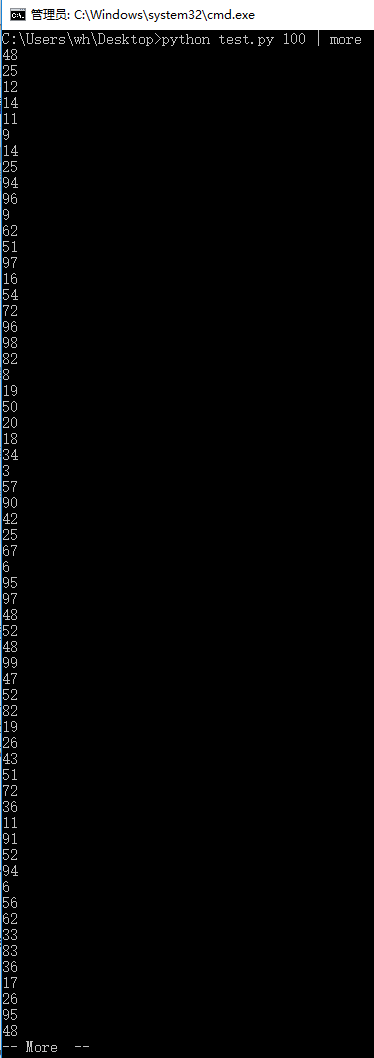使用操作系统实用程序sort排序输出数据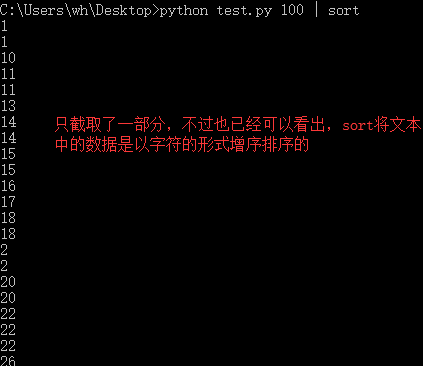more和sort都可以在一个语句中使用
填空题：1、2

print(value, ..., sep = ' ', end = '\n', file = sys.stdout, flush = False)
sep（分隔符，默认为空格）end（换行符，即输入的末尾是个啥）file（写入到指定文件流，默认为控制台sys.stdout）flush（指定是否强制写入到流）

1
>>> print(1,2,3,4,5,sep='-',end='!')
1-2-3-4-5!

2
>>> for i in range(10):
print(i,end=' ')

0 1 2 3 4 5 6 7 8 9

例题及上机实践：2~5
2. 尝试修改例6.2编写的命令行参数解析的程序，解析命令行参数所输入边长的值，计算并输出正方形的周长和面积

argparse模块用于解析命名的命令行参数，生成帮助信息的Python标准模块

例6.2：解析命令行参数所输入的长和宽的值，计算并输出长方形的面积
import argparse

parser = argparse.ArgumentParser()
parser.add_argument('--length', default = 10, type = int, help = '长度')
parser.add_argument('--width', default = 5, type = int, help = '宽度')

args = parser.parse_args()
area = args.length * args.width
print('面积 = ', area)

input()#加这一句是为了可以看到输出结果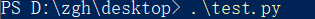输出：面积 = 50
如果在执行这个模块时，加入两个命令行参数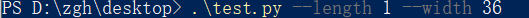输出：面积 = 36

基本上看了上面这个例子后，就可以理解argparse的用法了

本题代码：
import argparse

parser = argparse.ArgumentParser()
parser.add_argument('--length', default = 10, type = int, help = '长度')

args = parser.parse_args()
area = args.length ** 2
perimeter = 4 * args.length
print('面积 = {0}，周长 = {1}'.format(area,perimeter))

input()#加这一句是为了可以看到输出结果


在PowerShell中输入.\test.py 不给命令行参数，输出是以默认值来计算的 输出：面积 = 100，周长 = 40
给命令行参数：.\test.py --length 1 输出：面积 = 1，周长 = 4
3. 尝试修改例6.8编写读取并输出文本文件的程序，由命令行第一个参数确认所需输出的文本文件名

f = open(file, mode = 'r' , buffering = -1, encoding = None)
file是要打开或创建的文件名，如果文件不在当前路径，需指出具体路径mode是打开文件的模式，模式有： ‘r’（只读） ‘w’（写入，写入前删除就内容） ‘x’（创建新文件，如果文件存在，则导致FileExistsError） ‘a’（追加） ‘b’（二进制文件） ‘t’（文本文件，默认值） ‘+’（更新，读写）buffering表示是否使用缓存（缓存为-1，表示使用系统默认的缓冲区大小）encoding是文件的编码

例6.8：读取并输出文本文件
import sys

filename = sys.argv#就读取本文件，骚的呀皮
f = open(filename, 'r', encoding = 'utf-8')

line_no = 0
while True:
line_no += 1
if line:
print(line_no, ":", line)
else:
break
f.close()

输出（代码输出的就是本python文件）：
1 : import sys

2 :

3 : filename = sys.argv#就读取本文件，骚的呀皮

4 : f = open(filename, 'r', encoding = 'utf-8')

5 :

6 : line_no = 0

7 : while True:

8 :     line_no += 1

10 :     if line:

11 :         print(line_no, ":", line)

12 :     else:

13 :         break

14 : f.close()

15 :


本题代码：
对例题代码进行些许修改就可以了，首先将上例中的第二个语句改为：filename = sys.argv，再考虑下面怎么进行
准备一个用来测试的文件test.txt：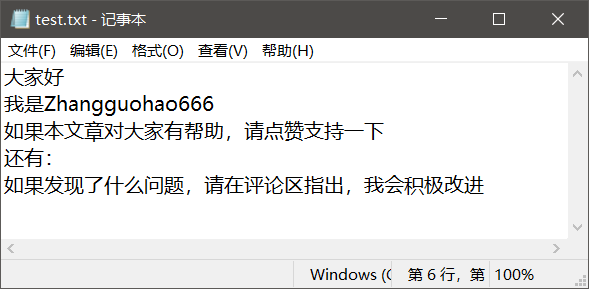对于这个文件要注意一点（你们很可能回出现这个问题！！！），win10默认创建的文本文件的字符编码是ANSI
代码怎么写，有两种：
将test.txt文本文件的编码修改为utf-8，代码如上所说 记事本方式打开test.txt文件，点击文件，点击另存为，看到下方的编码（修改为utf-8）test.txt就用默认的ANSI编码方式，再将上例代码的第三个语句修改为f = open(filename, 'r', encoding = 'ANSI')
在PowerShell中输入：./test.py test.txt 输出：
1 : ﻿大家好

2 : 我是Zhangguohao666

3 : 如果本文章对大家有帮助，请点赞支持一下

4 : 还有：

5 : 如果发现了什么问题，请在评论区指出，我会积极改进

4. 尝试修改例6.9编写利用with语句读取并输出文本文件的程序，由命令行第一个参数确认所需输出的文本文件名

为了简化操作，Python语言中与资源相关的对象可以实现上下文管理协议，可以使用with语句，确保释放资源。 with open(file,mode) as f:

例6.9：利用with语句读取并输出文本文件
import sys

filename = sys.argv

line_no = 0
with open(filename, 'r', encoding = 'utf-8') as f:
for line in f:
line_no += 1
print(line_no, ":", line)
f.close()


基本上，看这个例子，就可以上手with语句了

本题代码：
还是上一题准备的文本文件， 代码一（文本文件的编码为默认的ANSI）：
import sys

filename = sys.argv

line_no = 0
with open(filename, 'r', encoding = 'ANSI') as f:
for line in f:
line_no += 1
print(line_no, ":", line)
f.close()


代码二（将文本文件的编码修改为utf-8）：
import sys

filename = sys.argv

line_no = 0
with open(filename, 'r', encoding = 'utf-8') as f:
for line in f:
line_no += 1
print(line_no, ":", line)
f.close()


本题的输出，我再不要脸的放一次吧：
1 : ﻿大家好

2 : 我是Zhangguohao666

3 : 如果本文章对大家有帮助，请点赞支持一下

4 : 还有：

5 : 如果发现了什么问题，请在评论区指出，我会积极改进

5. 尝试修改例6.12编写标准输出流重定向的程序，从命令行第一个参数中获取n的值，然后将0-n，0-n的2倍值，2的0-n次幂的列表打印输出到out.log文件中
例6.12：从命令行第一个参数中获取n的值，然后将0-n，2的0-n次幂的列表打印输出到out.log文件中

标准输入流文件对象：sys.stdin， 默认值为sys.__stdin__标准输出流文件对象：sys.stdout， 默认值为sys.__stdout__错误输出流文件对象（标准错误流文件对象）：sys.stderr 默认值为sys.__stderr__

书中给的代码是这样的：
import sys

n = int(sys.argv)
power = 1
i = 0

f = open('out.log', 'w')
sys.stdout = f

while i <= n:
print(str(i), ' ', str(power))
power = 2*power
i += 1
sys.stdout = sys.__stdout__

如果使用的编辑器是PyCharm（现在大多数编辑器会帮你对代码进行优化和处理一些隐患），运行书中的这个代码没有问题。
但是： 若使用的编辑器是python自带的IDLE，运行这个代码有问题！
第一：out.log文件会生成，但是没有东西 （发现文件关闭不了（就是×不掉）， 确定是文件没关闭（f.close()）的原因）
第二：控制台没有输出’done’语句（估计是IDLE编辑器处理不了__stdout__这个值）
经过研究后，发现（基于IDLE编辑器）： 如果在上面的代码中加入f.close()后，该输入的东西都成功输入进out.log文件了， 但是， 还有一个问题 控制台依旧没有输出’done’语句 经过一步步的断点调试（就是手动写print） 发现sys.stdout = sys.__stdout__不会执行
然后进行改动后，就可以了，代码如下： （既然__stdout__不好使，就使用中间变量）
import sys

n = int(sys.argv)
power = 1
i = 0

output = sys.stdout
f = open('out.log', 'w')
sys.stdout = f

while i <= n:
print(str(i), ' ', str(power))
power = 2*power
i += 1

f.close()
sys.stdout = output
print('done!')#这一句是用来检测上面的代码是否成功执行



问题虽然解决，但是原因没有彻底弄清楚，求助。。。。。。

本题代码：
import sys

n = int(sys.argv)
power = 1
i = 0

output = sys.stdout
f = open('out.log', 'w')
sys.stdout = f

while i <= n:
print(str(i), ' ',  str(2*i),  ' ', str(power))
power = 2*power
i += 1

f.close()
sys.stdout = output
print('done!')#这一句是用来检测上面的代码是否成功执行


比如时输入的命令行参数是6 输出：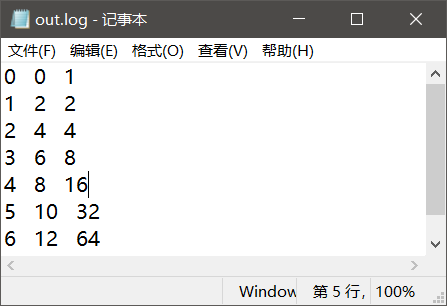案例研究：21点扑克牌游戏
https://blog.csdn.net/Zhangguohao666/article/details/103948545
通过21点扑克牌游戏的设计和实现，了解使用Python数据类型、控制流程和输入输出
第七章 错误和异常处理

Python语言采用结构化的异常处理机制捕获和处理异常

而我感觉，Python在这方面的知识点其实和Java的差不多
几个例题
一：程序的错误和异常处理
语法错误

指源代码中的拼写错误，这些错误导致Python编译器无法把Python源代码转换为字节码，故也称之为编译错误

运行时错误

在解释执行过程中产生的错误

例如：
程序中没有导入相关的模块，NameError程序中包括零除运算，ZeroDivisionError程序中试图打开不存在的文件，FileNotFoundError
逻辑错误

程序可以执行（程序运行本身不报错），但执行结果不正确。 对于逻辑错误，Python解释器无能为力，需要用户根据结果来调试判断

大部分由程序错误而产生的错误和异常一般由Python虚拟机自动抛出。另外，在程序中如果判断某种错误情况，可以创建相应的异常类的对象，并通过raise语句抛出
>>> a = -1
>>> if(a < 0): raise ValueError("数值不能为负数")

Traceback (most recent call last):
File "<pyshell#9>", line 1, in <module>
if(a < 0): raise ValueError("数值不能为负数")
ValueError: 数值不能为负数
>>>


在程序中的某个方法抛出异常后，Python虚拟机通过调用堆栈查找相应的异常捕获程序。如果找到匹配的异常捕获程序（即调用堆栈中的某函数使用try…except语句捕获处理），则执行相应的处理程序（try…except语句中匹配的except语句块）

如果堆栈中没有匹配的异常捕获程序，则Python虚拟机捕获处理异常，在控制台打印出异常的错误信息和调用堆栈，并中止程序的执行

二：try …except…else…finally
try:
可能产生异常的语句
except Exception1:
发生Exception1时执行的语句
except (Exception2,Exception3):
发生Exception2或Exception3时执行的语句
except Exception4 as e:
发生Exception4时执行的语句，Exception4的实例是e
except:
捕获其他所有异常
else:
无异常时执行的语句
finally:
不管异常发生与否都保证执行的语句

except语句可以写多个，但是要注意一点：系统是自上而下匹配发生的异常，所以用户需要将带有最具体的（即派生类程度最高的）异常类的except写在前面
三：创建自定义异常，处理应用程序中出现的负数参数的异常

自定义异常类一般继承于Exception或其子类。自定义异常类的名称一般以Error或Exception为后缀

>>> class NumberError(Exception):
def __init__(self,data):
Exception.__init__
(self,data)
self.data = data
def __str__(self):
return self.data + '：非法数值（<0）'

>>>
>>> def total(data):
total = 0
for i in data:
if i < 0: raise NumberError(str(i))
total += 1

>>>
>>> data1 = (44, 78, 90, 80, 55)
>>> print("sum: ",total(data1))
sum:  5
>>>
>>> data2 = (44, 78, 90, 80, -1)
>>> print("sum: ",total(data2))
Traceback (most recent call last):
File "<pyshell#24>", line 1, in <module>
print("sum: ",total(data2))
File "<pyshell#18>", line 4, in total
if i < 0: raise NumberError(str(i))
NumberError: -1：非法数值（<0）
>>>

四：断言处理
用户在编写程序时，在调试阶段往往需要判断代码执行过程中变量的值等信息：
用户可以使用print()函数打印输出结果也可以通过断点跟踪调试查看变量但使用断言更加灵活
assert语句和AssertionError
断言的声明：
assert <布尔表达式> 即：if __debug__: if not testexpression: raise AssertionErrorassert <布尔表达式>,<字符串表达式> 即：if __debug__: if not testexpression: raise AssertionError(data) 字符串表达式（即data）是断言失败时输出的失败消息
__debug__也是布尔值，Python解释器有两种：调试模式和优化模式
调试模式：__debug__ == True优化模式：__debug__ == False
在学习中，对于执行一个py模块（比如test.py）我们通常在cmd中这么输入python test.py，而这默认是调试模式。 如果我们要使用优化模式来禁用断言来提高程序效率，我们可以加一个运行选项-O，在控制台中这么输入python -O test.py
看一下断言的示例吧，理解一下用法：
a =int(input("a: "))
b =int(input("b: "))
assert b != 0, '除数不能为零'
c = a/b
print("a/b = ", c)

cmd出场： 输入正确数值时：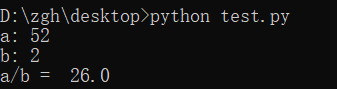输入错误数值时：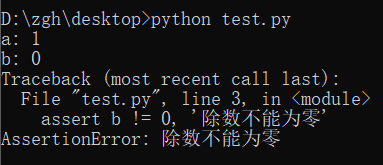禁用断言，并且输入错误数值时：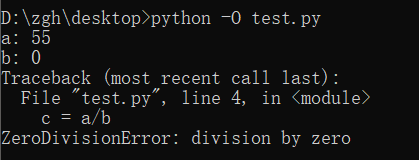案例研究：使用调试器调试Python程序
https://blog.csdn.net/Zhangguohao666/article/details/103948568
了解使用Python调试器调试程序的方法
第八章 函数和函数式编程

一些知识点总结和几个例题
Python中函数的分类：
内置函数 在程序中可以直接使用标准库函数 Python语言安装程序同时会安装若干标准库，例如math、random等第三方库函数 Python社区提供了许多其它高质量的库，在下载、安装这些库后，通过import语句可以导入库用户自定义函数
函数名为有效的标识符（命名规则为全小写字母，可以使用下划线增加可阅读性，例如my_func()）函数可以使用return返回值 如果函数体中包含return语句，则返回值， 否则不返回，即返回值为空（None），无返回值的函数相当于其它编程语言中的过程
调用函数之前程序必须先执行def语句，创建函数对象
内置函数对象会自动创建import导入模块时会执行模块中的def语句，创建模块中定义的函数Python程序结构顺序通常为import语句>函数定义>全局代码
一：产生副作用的函数，纯函数
打印等腰三角形
n = int(input("行数："))

def print_star(n):
print((" * " * n).center(50))

for i in range(1, 2*n, 2):
print_star(i)

输出：
行数：5
*
*  *  *
*  *  *  *  *
*  *  *  *  *  *  *
*  *  *  *  *  *  *  *  *

上面代码中的print_star()是一个产生副作用的函数，其副作用是向标准输出写入若干星号

副作用：例如读取键盘输入，产生输出，改变系统的状态等在一般情况下，产生副作用的函数相当于其它程序设计语言中的过程，可以省略return语句

定义计算并返回第n阶调和数（1+1/2+1/3+…+1/n）的函数，输出前n个调和数
def harmonic(n):
total = 0.0
for i in range(1, n+1):
total += 1.0/i

n = int(input("n："))

print("输出前n个调和数的值：")
for i in range(1, n+1):
print(harmonic(i))

输出：
 n：8
输出前n个调和数的值：
1.0
1.5
1.8333333333333333
2.083333333333333
2.283333333333333
2.4499999999999997
2.5928571428571425
2.7178571428571425

上面代码中的harmonic()是纯函数

纯函数：给定同样的实际参数，其返回值唯一，且不会产生其它的可观察到的副作用

注意：编写同时产生副作用和返回值的函数通常被认为是不良编程风格，但有一个例外，即读取函数。例如，input()函数既可以返回一个值，又可以产生副作用（从标准输入中读取并消耗一个字符串）
二：传递不可变对象、可变对象的引用
实际参数值默认按位置顺序依次传递给形式参数。如果参数个数不对，将会产生错误
在调用函数时：
若传递的是不可变对象（例如：int、float、bool、str对象）的引用，则如果函数体中修改对象的值，其结果实际上是创建了一个新的对象
i = 1

def func(i,n):
i += n
return i

print(i)#1
func(i,10)
print(i)#1

执行函数func()后，i依旧为1，而不是11
若传递的是可变对象（例如：list对象）的引用，则在函数体中可以直接修改对象的值
import random

def shuffle(a):
n = len(a)
for i in range(n):
r = random.randrange(i,n)
a[i],a[r] = a[r],a[i]

a = [1,2,3,4,5]
print("初始：",a)
shuffle(a)
print("调用函数后：",a)

输出：
初始： [1, 2, 3, 4, 5]
调用函数后： [1, 5, 4, 3, 2]

三：可选参数，命名参数，可变参数，强制命名参数
可选参数
在声明函数时，如果希望函数的一些参数是可选的，可以在声明函数时为这些参数指定默认值
>>> def babbles(words, times=1):
print(words * times)

>>> babbles('Hello')
Hello
>>>
>>> babbles("Hello", 2)
HelloHello
>>>

注意到一点：必须先声明没有默认值的形参，然后再声明有默认值的形参，否则报错。 这是因为在函数调用时默认是按位置传递实际参数的。
怎么理解上面那句话呢？
默认是按位置传递实际参数（如果有默认值的形参在左边，无默认值的形参在右，那么在调用函数时，你的实参该怎么传递呢？）
命名参数
位置参数：当函数调用时，实参默认按位置顺序传递形参命名参数（关键字参数）：按名称指定传入的参数 参数按名称意义明确 传递的参数与顺序无关 如果有多个可选参数，则可以选择指定某个参数值
基于期中成绩和期末成绩，按照指定的权重计算总评成绩
>>> def my_sum(mid_score, end_score, mid_rate = 0.4):
score = mid_score*mid_rate + end_score*(1-mid_rate)
print(format(score,'.2f'))

>>> my_sum(80,90)
86.00
>>> my_sum(mid_score = 80,end_score = 90)
86.00
>>> my_sum(end_score = 90,mid_score = 80)
86.00
>>>

可变参数
在声明函数时，可以通过带星号的参数（例如：def func(* param)）向函数传递可变数量的实参，调用函数时，从那一点后所有的参数被收集为一个元组在声明函数时，可以通过带双星号的参数（例如：def func(** param)）向函数传递可变数量的实参，调用函数时，从那一点后所有的参数被收集为一个字典
利用带星的参数计算各数字的累加和
>>> def my_sum(a,b,*c):
total = a+b
for i in c:
total += i

>>> print(my_sum(1,2))
3
>>> print(my_sum(1,2,3,4,5,6))
21

利用带星和带双星的参数计算各数字的累加和
>>> def my_sum(a,b,*c,**d):
total = a+b
for i in c:
total += i
for key in d:
total += d[key]

>>> print(my_sum(1,2))
3
>>> print(my_sum(1,2,3,4))
10
>>> print(my_sum(1,2,3,4,male=1,female=2))
13

强制命名参数
在带星号的参数后面声明参数会导致强制命名参数（Keyword-only），然后在调用时必须显式使用命名参数传递值因为按位置传递的参数默认收集为一个元组，传递给前面带星号的可变参数
>>> def my_sum(*, mid_score, end_score, mid_rate = 0.4):
score = mid_score*mid_rate + end_score*(1-mid_rate)
print(format(score,'.2f'))

>>> my_sum(mid_score=80,end_score=90)
86.00
>>> my_sum(end_score=90,mid_score=80)
86.00
>>> my_sum(80,90)
Traceback (most recent call last):
File "<pyshell#47>", line 1, in <module>
my_sum(80,90)
TypeError: my_sum() takes 0 positional arguments but 2 were given
>>>

四：全局语句global示例，非局部语句nonlocal示例，输出局部变量和全局变量
在函数体中可以引用全局变量，但是要为定义在函数外的全局变量赋值，需要使用global语句
pi = 2.1415926
e = 2.7182818

def my_func():
global pi
pi = 3.14
print("global pi = ", pi)
e = 2.718
print("local e = ", e)

print('module pi = ', pi)
print('module e = ', e)
my_func()
print('module pi = ', pi)
print('module e = ', e)

输出：
module pi =  2.1415926
module e =  2.7182818
global pi =  3.14
local e =  2.718
module pi =  3.14
module e =  2.7182818

在函数体中可以定义嵌套函数，在嵌套函数中如果要为定义在上级函数体的局部变量赋值，可以使用nonlocal
def outer_func():
tax_rate = 0.17
print('outer function tax rate is ',tax_rate)
def inner_func():
nonlocal tax_rate
tax_rate = 0.01
print('inner function tax rate is ',tax_rate)
inner_func()
print('outer function tax rate is ',tax_rate)

outer_func()

输出：
outer function tax rate is  0.17
inner function tax rate is  0.01
outer function tax rate is  0.01

输出局部变量和全局变量
内置函数locals()，局部变量列表内置函数globals()，全局变量列表
五：获取和设置最大递归数
在sys模块中，函数getrecursionlimit()和setrecursionlimit()用于获取和设置最大递归次数
>>> import sys
>>> sys.getrecursionlimit()
1000
>>> sys.setrecursionlimit(666)
>>> sys.getrecursionlimit()
666
>>>

六：三个有趣的内置函数：eval()、exec()、compile()
eval
对动态表达式进行求值，返回值eval(expression, globals=None, locals=None) expression是动态表达式的字符串 globals和locals是求值时使用的上下文环境的全局变量和局部变量，如果不指定，则使用当前运行上下文
>>> x = 2
>>> str_func = input("请输入表达式：")
请输入表达式：x**2+2*x+1
>>> eval(str_func)
9
>>>

exec
可以执行动态表达式，不返回值exec(str, globals=None, locals=None)
>>> exec("for i in range(10): print(i, end=' ')")
0 1 2 3 4 5 6 7 8 9
>>>

compile
编译代码为代码对象，可以提高效率compile(source, filename, mode) source为代码语句的字符串；如果是多行语句，则每一行的结尾必须有换行符\n filename为包含代码的文件 mode为编码方式，可以为'exec'（用于语句序列的执行），可以为'eval'（用于表达式求值），可以为'single'（用于单个交互语句）
>>> co = compile("for i in range(10): print(i, end=' ')", '', 'exec')
>>> exec(co)
0 1 2 3 4 5 6 7 8 9
>>>

七：map()，filter()
map(f, iterable,…)，将函数f应用于可迭代对象，返回结果为可迭代对象
示例1：
>>> def is_odd(x):
return x%2 == 1

>>> list(map(is_odd,range(5)))
[False, True, False, True, False]
>>>

示例2：
>>> list(map(abs,[1,-2,3,-4,5,-6]))
[1, 2, 3, 4, 5, 6]
>>>

示例3：
>>> list(map(str,[1,2,3,4,5]))
['1', '2', '3', '4', '5']
>>

示例4：
>>> def greater(x,y):
return x>y

>>> list(map(greater,[1,5,7,3,9],[2,8,4,6,0]))
[False, False, True, False, True]
>>>

filter(f, iterable)，将函数f应用于每个元素，然后根据返回值是True还是False决定保留还是丢弃该元素，返回结果为可迭代对象
示例1（返回个位数的奇数）：
>>> def is_odd(x):
return x%2 == 1

>>> list(filter(is_odd, range(10)))
[1, 3, 5, 7, 9]
>>>

示例2（返回三位数的回文）：
>>> list(filter(is_palindrome, range(100, 1000)))
[101, 111, 121, 131, 141, 151, 161, 171, 181, 191, 202, 212, 222, 232, 242, 252, 262, 272, 282, 292, 303, 313, 323, 333, 343, 353, 363, 373, 383, 393, 404, 414, 424, 434, 444, 454, 464, 474, 484, 494, 505, 515, 525, 535, 545, 555, 565, 575, 585, 595, 606, 616, 626, 636, 646, 656, 666, 676, 686, 696, 707, 717, 727, 737, 747, 757, 767, 777, 787, 797, 808, 818, 828, 838, 848, 858, 868, 878, 888, 898, 909, 919, 929, 939, 949, 959, 969, 979, 989, 999]
>>>

八：Lambda表达式和匿名函数

匿名函数广泛应用于需要函数对象作为参数、函数比较简单并且只使用一次的场合

格式：
lambda arg1,arg2... : <expression>

其中，arg1、arg2等为函数的参数，<expression>为函数的语句，其结果为函数的返回值
示例1（计算两数之和）：
>>> f = lambda x,y : x+y
>>> type(f)
<class 'function'>
>>> f(1,1)
2
>>>

示例2（返回奇数）：
>>> list(filter(lambda x:x%2==1, range(10)))
[1, 3, 5, 7, 9]
>>>

示例3（返回非空元素）：
>>> list(filter(lambda s:s and s.strip(), ['A', '', 'B', None, 'C', ' ']))
['A', 'B', 'C']
>>>

补充：
strip()用来去除头尾字符、空白符（\n,\r,\t,’’,即换行、回车、制表、空格）lstrip()用来去除开头字符、空白符rstrip()用来去除结尾字符、空白符
再补充一点：
\n到下一行的开头\r回到这一行的开头
示例4（返回大于0的元素）：
>>> list(filter(lambda x:x>0, [1,0,-2,8,5]))
[1, 8, 5]
>>>

示例5（返回元素的平方）：
>>> list(map(lambda x:x*x, range(10)))
[0, 1, 4, 9, 16, 25, 36, 49, 64, 81]
>>>

九：operator模块和操作符函数

Python内置操作符的函数接口，它定义了对应算术和比较等操作的函数，用于map()、filter()等需要传递函数对象作为参数的场合，可以直接使用而不需要使用函数定义或者Lambda表达式，使得代码更加简洁

示例1（concat(x,y)对应于x+y）：
>>> import operator
>>> a = 'hello'
>>>> operator.concat(a, ' world')
'hello world'

实例2（operator.gt对应于操作符>）：
>>> import operator
>>> list(map(operator.gt, [1,5,7,3,9],[2,8,4,6,0]))
[False, False, True, False, True]
>>>

十：functools.reduce()，偏函数functools.partial()，sorted()
functools.reduce()

functools.reduce(func, iterable[, iterable[, initializer]])
使用指定的带两个参数的函数func对一个数据集合的所有数据进行下列操作：使用第一个和第二个数据作为参数用func()函数运算，得到的结果再与第三个数据作为参数用func()函数运算，依此类推，最后得到一个结果可选的initialzer为初始值

示例：
>>> import functools,operator
15
25
5050
>>>
>>> functools.reduce(operator.mul, range(1,11))
3628800

偏函数functools.partial()

functools.partial(func, *arg, **keywords)
通过把一个函数的部分参数设置为默认值的方式返回一个新的可调用（callable）的partial对象主要用于设置预先已知的参数，从而减少调用时传递参数的个数

示例（2的n次方）：
>>> import functools,math
>>> pow2 = functools.partial(math.pow, 2)
>>> list(map(pow2, range(11)))
[1.0, 2.0, 4.0, 8.0, 16.0, 32.0, 64.0, 128.0, 256.0, 512.0, 1024.0]
>>>

十一：sorted()

sorted(iterable, *, key=None, reverse=False)
iterable是待排序的可迭代对象key是比较函数（默认为None，按自然顺序排序）reverse用于指定是否逆序排序

示例1（数值。默认自然排序）：
>>> sorted([1,6,4,-2,9])
[-2, 1, 4, 6, 9]
>>> sorted([1,6,4,-2,9], reverse=True)
[9, 6, 4, 1, -2]
>>> sorted([1,6,4,-2,9], key=abs)
[1, -2, 4, 6, 9]

示例2（字符串，默认按字符串字典序排序）：
>>> sorted(['Dod', 'cat', 'Rabbit'])
['Dod', 'Rabbit', 'cat']
>>> sorted(['Dod', 'cat', 'Rabbit'], key=str.lower)
['cat', 'Dod', 'Rabbit']
>>> sorted(['Dod', 'cat', 'Rabbit'], key=len)
['Dod', 'cat', 'Rabbit']

示例3（元组，默认按元组的第一个元素排序）：
>>> sorted([('Bob', 75), ('Adam', 92), ('Lisa', 88)])
[('Adam', 92), ('Bob', 75), ('Lisa', 88)]
>>> sorted([('Bob', 75), ('Adam', 92), ('Lisa', 88)], key=lambda t:t)
[('Bob', 75), ('Lisa', 88), ('Adam', 92)]

十二：函数装饰器
这玩意就很有意思了，很Java语言中的注解是很相像的
示例1：
import time,functools

def timeit(func):
def wrapper(*s):
start = time.perf_counter()
func(*s)
end = time.perf_counter()
print('运行时间：', end - start)
return wrapper

@timeit
def my_sum(n):
sum = 0
for i in range(n): sum += i
print(sum)

if __name__ == '__main__':
my_sum(10_0000)

结果：
4999950000
运行时间： 0.013929100000000028

怎么理解上面的代码呢？
首先，timeit()返回的是wrapper，而不是执行（没有小括号）@timeit相当于，在调用my_sum()的前一刻，会执行这么个语句：my_sum = timeit(my_sum)
示例2：
def makebold(fn):
def wrapper(*s):
return "<b>" + fn(*s) + "</b>"
return wrapper

def makeitalic(fn):
def wrapper(*s):
return "<i>" + fn(*s) + "</i>"
return wrapper

@makebold
@makeitalic
def htmltags(str1):
return str1

print(htmltags('Hello'))


输出：
<b><i>Hello</i></b>

选择题：1~5
1
>>> print(type(lambda:None))
<class 'function'>

2
>>> f = lambda x,y:x*y
>>> f(12, 34)
408

3
>>> f1 = lambda x:x*2
>>> f2 = lambda x:x**2
>>> print(f1(f2(2)))
8

4
>>> def f1(p, **p2):
print(type(p2))

>>> f1(1, a=2)
<class 'dict'>

5
>>> def f1(a,b,c):
print(a+b)

>>> nums = (1,2,3)
>>> f1(*nums)
3

思考题：4~11
4
>>> d = lambda p:p*2
>>> t = lambda p:p*3
>>> x = 2
>>> x = d(x)
>>> x = t(x)
>>> x = d(x)
>>> print(x)
24

5
>>> i = map(lambda x:x**2, (1,2,3))
>>> for t in i:
print(t, end=' ')

1 4 9

6
>>> def f1():
"simple function"
pass

>>> print(f1.__doc__)
simple function

7
>>> counter = 1
>>> num = 0
>>> def TestVariable():
global counter
for i in (1, 2, 3) : counter += 1
num = 10

>>> TestVariable()
>>> print(counter, num)
4 0

8
>>> def f(a,b):
if b==0 : print(a)
else : f(b, a%b)

>>> print(f(9,6))
3
None

求最大公约数
9
>>> def aFunction():
"The quick brown fox"
return 1

>>> print(aFunction.__doc__[4:9])
quick

10
>>> def judge(param1, *param2):
print(type(param2))
print(param2)

>>> judge(1, 2, 3, 4, 5)
<class 'tuple'>
(2, 3, 4, 5)

11
>>> def judge(param1, **param2):
print(type(param2))
print(param2)

>>> judge(1, a=2, b=3, c=4, d=5)
<class 'dict'>
{'a': 2, 'b': 3, 'c': 4, 'd': 5}

上机实践：2~5
2. 编写程序，定义一个求阶乘的函数fact(n)，并编写测试代码，要求输入整数n（n>=0）。请分别使用递归和非递归方式实现
递归方式：
def fact(n):
if n == 0 :
return 1
return n*fact(n-1)

n = int(input("请输入整数n（n>=0）："))
print(str(n)+" ! =  " + str(fact(n)))


非递归方式：
def fact(n):
t = 1
for i in range(1,n+1):
t *= i
return t

n = int(input("请输入整数n（n>=0）："))
print(str(n)+" ! =  " + str(fact(n)))


输出：
请输入整数n（n>=0）：5
5 ! =  120

3. 编写程序，定义一个求Fibonacci数列的函数fib(n)，并编写测试代码，输出前20项（每项宽度5个字符位置，右对齐），每行输出10个。请分别使用递归和非递归方式实现
递归方式：
def fib(n):
if (n == 1 or n == 2):
return 1
return fib(n-1)+fib(n-2)

for i in range(1,21):
print(str(fib(i)).rjust(5,' '),end = ' ')
if i %10 == 0:
print()

非递归方式：
def fib(n):
if (n == 1 or n == 2):
return 1
n1 = n2 = 1
for i  in range(3,n+1):
n3 = n1+n2
n1 = n2
n2 = n3
return n3

for i in range(1,21):
print(str(fib(i)).rjust(5,' '),end = ' ')
if i %10 == 0:
print()

输出：
    1     1     2     3     5     8    13    21    34    55
89   144   233   377   610   987  1597  2584  4181  6765

4. 编写程序，利用可变参数定义一个求任意个数数值的最小值的函数min_n(a,b,*c)，并编写测试代码。例如对于“print(min_n(8, 2))”以及“print(min_n(16, 1, 7, 4, 15))”的测试代码
def min_n(a,b,*c):
min_number = a if(a < b) else b
for n in c:
if n < min_number:
min_number = n
return min_number

print(min_n(8, 2))
print(min_n(16, 1, 7, 4, 15))

输出：
2
1

5. 编写程序，利用元组作为函数的返回值，求序列类型中的最大值、最小值和元素个数，并编写测试代码，假设测试代码数据分别为s1=[9, 7, 8, 3, 2, 1, 55, 6]、s2=[“apple”, “pear”, “melon”, “kiwi”]和s3="TheQuickBrownFox"
def func(n):
return (max(n),min(n),len(n))

s1=[9, 7, 8, 3, 2, 1, 55, 6]
s2=["apple", "pear", "melon", "kiwi"]
s3="TheQuickBrownFox"

for i in (s1,s2,s3):
print("list = ", i)
t = func(i)
print("最大值 = {0}，最小值 = {1}，元素个数 = {2}".format(t, t, t))

输出：
list =  [9, 7, 8, 3, 2, 1, 55, 6]
最大值 = 55，最小值 = 1，元素个数 = 8
list =  ['apple', 'pear', 'melon', 'kiwi']
最大值 = pear，最小值 = apple，元素个数 = 4
list =  TheQuickBrownFox
最大值 = x，最小值 = B，元素个数 = 16

案例研究：井字棋游戏
https://blog.csdn.net/Zhangguohao666/article/details/103280740
了解Python函数的定义和使用

由于本文的内容太多了，导致了两个很不好的结果， 一是：在网页中打开本篇博客的加载时间太长了，明显的卡顿很影响阅读体验； 二是：本人在对本篇文章进行更新或者修改内容时，卡的要死。 遂， 将本文第八章后面的很多内容拆分到新的文章中，望大家理解

第九章 面向对象的程序设计

《Python程序设计与算法基础教程（第二版）》江红 余青松，第九章课后习题答案
第十章 模块和客户端

《Python程序设计与算法基础教程（第二版）》江红 余青松，第十章课后习题答案
第十一章 算法与数据结构基础

《Python程序设计与算法基础教程（第二版）》江红 余青松，第十一章课后习题答案
第十二章 图形用户界面

我对图形用户界面基本无兴趣，无特殊情况，基本不打算碰这方面内容
案例研究：简易图形用户界面计算器
Python实现简易图形用户界面计算器
第十三章 图形绘制

与上一章相同，我对于图形绘制的兴趣也基本没有，尝试做了2-7题，就完全没兴趣做下去了
图形绘制模块：tkinter
2. 参考例13.2利用Canvas组件创建绘制矩形的程序，尝试改变矩形边框颜色以及填充颜色
from tkinter import *

root = Tk()
c = Canvas(root, bg = 'white', width = 130, height = 70)
c.pack()

c.create_rectangle(10, 10, 60, 60, fill = 'red')
c.create_rectangle(70, 10, 120, 60, fill = 'green', outline = 'blue', width = 5)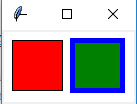创建画布对象：
root = Tk() 创建一个Tk根窗口组件rootc = Canvas(root, bg = 'white', width = 130, height = 70) 创建大小为200 * 100、背景颜色为白色的画布c.pack() 调用组件pack()方法，调整其显示位置和大小
绘制矩形：
c.create_rectangle(x0, y0, x1, y1, option, ...)

（x0，y0）是左上角的坐标（x1，y1）是右下角的坐标c.create_rectangle(70, 10, 120, 60, fill = 'green', outline = 'blue', width = 5) 用蓝色边框、绿色填充矩形，边框宽度为5
3. 参考例13.3利用Canvas组件创建绘制椭圆的程序，尝试修改椭圆边框样式、边框颜色以及填充颜色
from tkinter import *

root = Tk()
c = Canvas(root, bg = 'white', width = 280, height = 70)
c.pack()

c.create_oval(10, 10, 60, 60, fill = 'green')
c.create_oval(70, 10, 120, 60, fill = 'green', outline = 'red', width = 5)
c.create_oval(130, 25, 180, 45, dash = (10,))
c.create_oval(190, 10, 270, 50, dash = (1,), width = 2)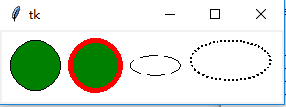绘制椭圆
c.create_oval(x0, y0, x1, y1, option, ...)

（x0，y0）是左上角的坐标（x1，y1）是右下角的坐标c.create_oval(70, 10, 120, 60, fill = 'green', outline = 'red', width = 5) 绿色填充、红色边框，宽度为5c.create_oval(130, 25, 180, 45, dash = (10,)) 虚线椭圆
4. 参考例13.4利用Canvas组件创建绘制圆弧的程序，尝试修改圆弧样式、边框颜色以及填充颜色
from tkinter import *

root = Tk()
c = Canvas(root, bg = 'white', width = 250, height = 70)
c.pack()

c.create_arc(10, 10, 60, 60, style = ARC)
c.create_arc(70, 10, 120, 60, style = CHORD)
c.create_arc(130, 10, 180, 60, style = PIESLICE)
for i in range(0, 360, 60):
c.create_arc(190, 10, 240, 60, fill = 'green', outline = 'red', start = i, extent = 30)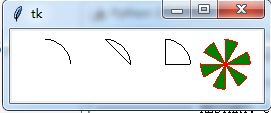绘制圆弧：
c.create_arc(x0, y0, x1, y1, option, ...)

（x0，y0）是左上角的坐标（x1，y1）是右下角的坐标选项start（开始角度，默认为0）和extend（圆弧角度，从start开始逆时针旋转，默认为90度）决定圆弧的角度范围选项start用于设置圆弧的样式
5. 参考例13.5利用Canvas组件创建绘制线条的程序，尝试修改线条样式和颜色
from tkinter import *

root = Tk()
c = Canvas(root, bg = 'white', width = 250, height = 70)
c.pack()

c.create_line(10, 10, 60, 60, arrow = BOTH, arrowshape = (3, 4, 5))
c.create_line(70, 10, 95, 10, 120, 60, fill = 'red')
c.create_line(130, 10, 180, 10, 130, 60, 180, 60, fill = 'green', width = 10, arrow = BOTH, joinstyle = MITER)
c.create_line(190, 10, 240, 10, 190, 60, 240, 60, width = 10)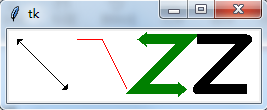绘制线条：
c.create_line(x0, y0, x1, y1, ..., xn, yn, option, ...)

（x0，y0）,（x1，y1），…，（xn，yn）是线条上各个点的坐标
6. 参考例13.6利用Canvas组件创建绘制多边形的程序，尝试修改多边形的形状、线条样式和填充颜色
from tkinter import *

root = Tk()
c = Canvas(root, bg = 'white', width = 250, height = 70)
c.pack()

c.create_polygon(35, 10, 10, 60, 60, 60, fill = 'red', outline = 'green')
c.create_polygon(70, 10, 120, 10, 120, 60, fill = 'white', outline = 'blue')
c.create_polygon(130, 10, 180, 10, 180, 60, 130, 60, outline = 'blue')
c.create_polygon(190, 10, 240, 10, 190, 60, 240, 60, fill = 'white', outline = 'black')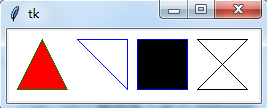绘制多边形：
c.create_polygon(x0, y0, x1, y1, ..., option, ...)

（x0，y0）,（x1，y1），…，（xn，yn）是多边形上各个顶点的坐标
7. 参考例13.7利用Canvas组件创建绘制字符串和图形的程序，绘制y = cos(x) 的图形
绘制字符串：
c.create_text(x, y, option, ...)

（x，y）是字符串放置的中心位置
y = sin(x)
from tkinter import *
import math

WIDTH, HEIGHT = 510, 210
ORIGIN_X, ORIGIN_Y = 2, HEIGHT/2 #原点

SCALE_X, SCALE_Y = 40, 100 #x轴、y轴缩放倍数
ox, oy = 0, 0
x, y = 0, 0
arc = 0 #弧度
END_ARC = 360 * 2 #函数图形画两个周期

root = Tk()
c = Canvas(root, bg = 'white', width = WIDTH, height = HEIGHT)
c.pack()

c.create_text(200, 20, text = 'y = sin(x)')
c.create_line(0, ORIGIN_Y, WIDTH, ORIGIN_Y)
c.create_line(ORIGIN_X, 0, ORIGIN_X, HEIGHT) #绘制x轴，y轴
for i in range(0, END_ARC+1, 10):
arc = math.pi * i / 180
x = ORIGIN_X + arc * SCALE_X
y = ORIGIN_Y - math.sin(arc) * SCALE_Y
c.create_line(ox, oy, x, y)
ox, oy = x, y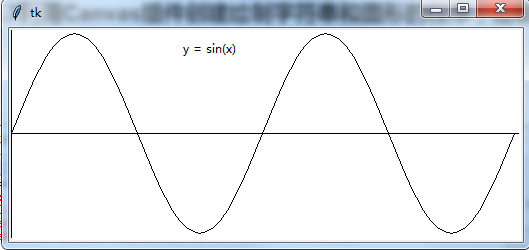y = cos(x)
from tkinter import *
import math

WIDTH, HEIGHT = 510, 210
ORIGIN_X, ORIGIN_Y = 2, HEIGHT/2 #原点

SCALE_X, SCALE_Y = 40, 100 #x轴、y轴缩放倍数
ox, oy = 0, 0
x, y = 0, 0
arc = 0 #弧度
END_ARC = 360 * 2 #函数图形画两个周期

root = Tk()
c = Canvas(root, bg = 'white', width = WIDTH, height = HEIGHT)
c.pack()

c.create_text(200, 20, text = 'y = cos(x)')
c.create_line(0, ORIGIN_Y, WIDTH, ORIGIN_Y)
c.create_line(ORIGIN_X, 0, ORIGIN_X, HEIGHT)
for i in range(0, END_ARC+1, 10):
arc = math.pi * i / 180
x = ORIGIN_X + arc * SCALE_X
y = ORIGIN_Y - math.cos(arc) * SCALE_Y
c.create_line(ox, oy, x, y)
ox, oy = x, y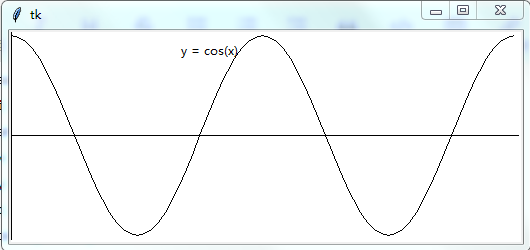图形绘制模块：turtle
…

后面章节内容：未完待续…
第十四章 数值日期和时间处理

第十五章 字符串和文本处理

第十六章 文件和数据交换

第十七章 数据访问

第十八章 网络编程和通信

第十九章 并行计算：进程、线程和协程

第二十章 系统管理
展开全文mfc api 汇编
• ## 前端面试题

万次阅读 多人点赞 2019-08-08 11:49:01
、HTML和CSS 21 你做的页面在哪些流览器测试过？这些浏览器的内核分别是什么? 21 每HTML文件里开头都有很重要的东西，Doctype，知道这是干什么的吗？ 21 Quirks模式是什么？它和Standards模式有什么区别 21 ...前端面试题
• ## 前端面试锦集

千次阅读 多人点赞 2019-07-20 13:41:45
使用立即执行函数的好处：通过定义一个匿名函数，创建一个新的函数作用域，相当于创建一个“私有”的命名空间，该命名空间的变量和方法，不会破坏污染全局的命名空间。此时若是想访问全局对象，将全局对象以参数...前端面试
• 章 C#语言基础 本章介绍C#语言的基础知识，希望具有C语言的读者能够基本掌握C#语言，并以此为基础，能够进一步学习用C#语言编写window应用程序和Web应用程序。当然仅靠章的内容就完全掌握C#语言是不可能的，...c#
• ## mybatis

千次阅读 2019-06-28 13:27:05
mybatis第天 1.mybatis概述和环境搭建 mybatis概述 mybatis环境搭建 1. 创建maven工程、添加开发依赖、创建数据库和表； 2. 创建domain实体类和dao mybatis是一门java语言编写持久层框架，大大简化了...mybatis
• ## 测试开发笔记

万次阅读 多人点赞 2019-11-14 17:11:58
章 测试基础 7 什么是软件测试： 7 ★软件测试的目的、意义：（怎么做好软件测试） 7 3.软件生命周期： 7 第二章 测试过程 8 1.测试模型 8 H模型： 8 V模型 9 2.内部测试 10 3外部测试： 10 验收测试：（在系统...测试开发
• ## JAVA上百实例源码以及开源项目

千次下载 热门讨论 2016-01-03 17:37:40
用JAVA开发的一个小型的目录监视系统，系统会每5秒自动扫描一次需要监视的目录，可以用来监视目录文件大小及文件增减数目的变化。 Java日期选择控件完整源代码 14个目标文件 内容索引:JAVA源码,系统相关,日历,...
• ## VC创建线程

千次阅读 2018-11-07 20:42:29
• ## Java笔试面试题整理第八波

万次阅读 多人点赞 2016-06-14 11:07:41
本系列整理Java相关的笔试面试知识点，其他几篇文章如下：  Java笔试面试题整理第七波  Java笔试面试题整理第六波 ... Java笔试面试题整理第波 1、运算符相关 What results from the fJava面试题 Java笔试题 泛型问题 变量初始化
• 答：缺省端口是8080，若要修改，可以进入Tomcat的安装目录下找到conf目录下的server.xml文件，找到该文件的Connector字段的port。 2、Tomcat有哪几种connector运行模式（服务的请求方式）？ 答：三种。修改它的...Tomcat面试题 Nginx面试题
• ## WPF开发教程

万次阅读 多人点赞 2019-07-02 23:13:20
WPF 的每个 Visual 可以创建组合节点，也可以创建一个或多个组合节点。 请注意一个非常重要的体系结构细节 – 可视对象和绘制指令的整个树都要进行缓存。在图形方面，WPF 使用一个保留的呈现系统。这可以...WPF
• ## C#线程（一）概述及常用方法

千次阅读 多人点赞 2018-05-12 12:47:20C#
• 2. 下列方法中可以用来创建一个新线程的是（AD） A. 实现 java.lang.Runnable 接口并重写 run () 方法 B. 实现 java.lang.Runnable 接口并重写 start() 方法 C. 实现 java.lang.Thread 类并实现 start() ...
• c语言库 process.h 的函数， 用来创建一个线程 unsignedlong_beginthreadex( void*security,//安全属性，为NULL时表示默认安全性 unsignedstack_size,//线程的堆栈大小，一般默认为0 ....
• 3.线程可以用yield方法使低优先级的线程运行。（ F ） yield方法：暂停线程，暂停当前正在执行的线程对象。yield()只是使当前线程重新回到可执行状态，所以执行yield()的线程有可能在进入到可执行状态后马上又被执行...java eclipse
• java面试题整理（3） JAVA常考点3 目录 1. 讲下JAVA的运行时区域 1 2、简单说下垃圾回收机制 2 ...5、 MySQL存储引擎的MyISAM和InnoDB区别 7 6、B树与B+树简明扼要的区别 11 6、 解决哈希冲突的三种...java面试
• 因此我们必须要重新开启一个后台线程运行这些任务。然而，往往这些任务最终又会直接或者间接的需要访问和控制UI控件。例如访问网络获取数据，然后需要将这些数据处理显示出来。就出现了上面所说的android 交互设计 控件
• ## 多线程作业及答案

千次阅读 2020-04-05 15:59:04
线程作业 一、填空题 1.处于运行状态的线程在某些情况下，如执行了sleep（睡眠）方法，或等待I/O设备等资源，将让出CPU并暂时停止自己的运行，进入_________状态。...3.一个正在执行的线程可能被人为地中断，让...
• 在多线程环境下，我们可能会需要等待开辟的线程执行完后，再去执行某个方法，例如输出并行计算结果等。 但是在多线程下，线程的执行是不阻塞主线程的，这点其实也是多线程的优势，提高代码执行效率，不必相互等待...
• java允许多线程并发控制，当多线程同时操作一个可共享的资源变量时，将会导致数据不准确，相互之间产生冲突，因此加入同步锁以避免在该线程没有完成操作之前，被其他线程调用，从而保证了该变量的唯一性和准确性 ...
• vector：就比arraylist多了同步化机制（线程安全），因为效率较低，现在已经不太建议使用。在web应用，特别是前台页面，往往效率（页面响应速度）是优先考虑的。 statck：堆栈类，先进后出 hashtable：就比...
• 本文转载自：详解阿里Java面试题——多线程精选53题【含答案】，面试必备 前言 为了能够在面试回答优雅而不失体面回答面试考点，该文章借鉴了不同平台对知识点的描述。 如有侵权请联系我 文章的不足和错误请指正...Java 面试题 程序人生 架构师成长之路
• 有没有可能发生其他两种转换一个或两个？ 答：从阻塞到运行的转换是可以想象的。假设某个进程在I/O上阻塞，而且I/O结束，如果此时CPU空闲，该进程就可以从阻塞态直接转到运行态。而另外一种转换（从阻塞态...操作系统 cpu 进程
• 3. 同一个进程或另一个进程线程调用TerminateThread 函数（最好避免使用这种方法 ）。 4. 该线程的主进程终止运行（避免使用 ）。 下面将介绍终止线程运行的方法，并且说明线程终止运行
• ## C#多线程和异步

万次阅读 多人点赞 2018-04-25 22:12:43
、使用计时器在某些情况下，可能不需要使用单独的线程。如果应用程序需要定期执行简单的与 UI 有关的操作，则应该考虑使用进程计时器。有时，在智能客户端应用程序使用进程计时器，以达到下列目：• 按计划定期...异步...